$$\newcommand{\half}{\frac{1}{2}} \newcommand{\halfi}{{1/2}} \newcommand{\tp}{\thinspace .} \newcommand{\uex}{{u_{\small\mbox{e}}}} \newcommand{\uexd}{{u_{\small\mbox{e}, #1}}} \newcommand{\vex}{{v_{\small\mbox{e}}}} \newcommand{\Aex}{{A_{\small\mbox{e}}}} \newcommand{\E}{\hbox{E}\lbrack #1 \rbrack} \newcommand{\Var}{\hbox{Var}\lbrack #1 \rbrack} \newcommand{\xpoint}{\boldsymbol{x}} \newcommand{\normalvec}{\boldsymbol{n}} \newcommand{\Oof}{\mathcal{O}(#1)} \newcommand{\x}{\boldsymbol{x}} \renewcommand{\u}{\boldsymbol{u}} \renewcommand{\v}{\boldsymbol{v}} \newcommand{\acc}{\boldsymbol{a}} \newcommand{\rpos}{\boldsymbol{r}} \newcommand{\e}{\boldsymbol{e}} \newcommand{\f}{\boldsymbol{f}} \newcommand{\F}{\boldsymbol{F}} \newcommand{\stress}{\boldsymbol{\sigma}} \newcommand{\I}{\boldsymbol{I}} \newcommand{\T}{\boldsymbol{T}} \newcommand{\q}{\boldsymbol{q}} \newcommand{\g}{\boldsymbol{g}} \newcommand{\dfc}{\alpha} % diffusion coefficient \newcommand{\ii}{\boldsymbol{i}} \newcommand{\jj}{\boldsymbol{j}} \newcommand{\kk}{\boldsymbol{k}} \newcommand{\ir}{\boldsymbol{i}_r} \newcommand{\ith}{\boldsymbol{i}_{\theta}} \newcommand{\Ix}{\mathcal{I}_x} \newcommand{\Iy}{\mathcal{I}_y} \newcommand{\Iz}{\mathcal{I}_z} \newcommand{\It}{\mathcal{I}_t} \newcommand{\setb}{#1^0} % set begin \newcommand{\sete}{#1^{-1}} % set end \newcommand{\setl}{#1^-} \newcommand{\setr}{#1^+} \newcommand{\seti}{#1^i} \newcommand{\stepzero}{*} \newcommand{\stephalf}{***} \newcommand{\stepone}{**} \newcommand{\baspsi}{\psi} \newcommand{\dx}{\, \mathrm{d}x} \newcommand{\ds}{\, \mathrm{d}s} \newcommand{\Real}{\mathbb{R}} \newcommand{\Integer}{\mathbb{Z}}$$

# Vibration ODEs

Vibration problems lead to differential equations with solutions that oscillate in time, typically in a damped or undamped sinusoidal fashion. Such solutions put certain demands on the numerical methods compared to other phenomena whose solutions are monotone or very smooth. Both the frequency and amplitude of the oscillations need to be accurately handled by the numerical schemes. The forthcoming text presents a range of different methods, from classical ones (Runge-Kutta and midpoint/Crank-Nicolson methods), to more modern and popular symplectic (geometric) integration schemes (Leapfrog, Euler-Cromer, and Stoermer-Verlet methods), but with a clear emphasis on the latter. Vibration problems occur throughout mechanics and physics, but the methods discussed in this text are also fundamental for constructing successful algorithms for partial differential equations of wave nature in multiple spatial dimensions.

# Finite difference discretization

Many of the numerical challenges faced when computing oscillatory solutions to ODEs and PDEs can be captured by the very simple ODE $$u^{\prime\prime} + u =0$$. This ODE is thus chosen as our starting point for method development, implementation, and analysis.

## A basic model for vibrations

The simplest model of a vibrating mechanical system has the following form: $$\begin{equation} u^{\prime\prime} + \omega^2u = 0,\quad u(0)=I,\ u^{\prime}(0)=0,\ t\in (0,T] \tp \tag{1.1} \end{equation}$$ Here, $$\omega$$ and $$I$$ are given constants. The section Oscillating mass attached to a spring derives (1.1) from physical principles and explains what the constants mean.

The exact solution of (1.1) is $$\begin{equation} u(t) = I\cos (\omega t) \tp \tag{1.2} \end{equation}$$ That is, $$u$$ oscillates with constant amplitude $$I$$ and angular frequency $$\omega$$. The corresponding period of oscillations (i.e., the time between two neighboring peaks in the cosine function) is $$P=2\pi/\omega$$. The number of periods per second is $$f=\omega/(2\pi)$$ and measured in the unit Hz. Both $$f$$ and $$\omega$$ are referred to as frequency, but $$\omega$$ is more precisely named angular frequency, measured in rad/s.

In vibrating mechanical systems modeled by (1.1), $$u(t)$$ very often represents a position or a displacement of a particular point in the system. The derivative $$u^{\prime}(t)$$ then has the interpretation of velocity, and $$u^{\prime\prime}(t)$$ is the associated acceleration. The model (1.1) is not only applicable to vibrating mechanical systems, but also to oscillations in electrical circuits.

## A centered finite difference scheme

To formulate a finite difference method for the model problem (1.1) we follow the four steps explained in .

### Step 1: Discretizing the domain

The domain is discretized by introducing a uniformly partitioned time mesh. The points in the mesh are $$t_n=n\Delta t$$, $$n=0,1,\ldots,N_t$$, where $$\Delta t = T/N_t$$ is the constant length of the time steps. We introduce a mesh function $$u^n$$ for $$n=0,1,\ldots,N_t$$, which approximates the exact solution at the mesh points. (Note that $$n=0$$ is the known initial condition, so $$u^n$$ is identical to the mathematical $$u$$ at this point.) The mesh function $$u^n$$ will be computed from algebraic equations derived from the differential equation problem.

### Step 2: Fulfilling the equation at discrete time points

The ODE is to be satisfied at each mesh point where the solution must be found: $$\begin{equation} u^{\prime\prime}(t_n) + \omega^2u(t_n) = 0,\quad n=1,\ldots,N_t \tp \tag{1.3} \end{equation}$$

### Step 3: Replacing derivatives by finite differences

The derivative $$u^{\prime\prime}(t_n)$$ is to be replaced by a finite difference approximation. A common second-order accurate approximation to the second-order derivative is $$\begin{equation} u^{\prime\prime}(t_n) \approx \frac{u^{n+1}-2u^n + u^{n-1}}{\Delta t^2} \tp \tag{1.4} \end{equation}$$ Inserting (1.4) in (1.3) yields $$\begin{equation} \frac{u^{n+1}-2u^n + u^{n-1}}{\Delta t^2} = -\omega^2 u^n \tp \tag{1.5} \end{equation}$$

We also need to replace the derivative in the initial condition by a finite difference. Here we choose a centered difference, whose accuracy is similar to the centered difference we used for $$u^{\prime\prime}$$: $$\begin{equation} \frac{u^1-u^{-1}}{2\Delta t} = 0 \tag{1.6} \tp \end{equation}$$

### Step 4: Formulating a recursive algorithm

To formulate the computational algorithm, we assume that we have already computed $$u^{n-1}$$ and $$u^n$$, such that $$u^{n+1}$$ is the unknown value to be solved for: $$\begin{equation} u^{n+1} = 2u^n - u^{n-1} - \Delta t^2\omega^2 u^n \tp \tag{1.7} \end{equation}$$ The computational algorithm is simply to apply (1.7) successively for $$n=1,2,\ldots,N_t-1$$. This numerical scheme sometimes goes under the name Stoermer's method, Verlet integration, or the Leapfrog method (one should note that Leapfrog is used for many quite different methods for quite different differential equations!).

### Computing the first step

We observe that (1.7) cannot be used for $$n=0$$ since the computation of $$u^1$$ then involves the undefined value $$u^{-1}$$ at $$t=-\Delta t$$. The discretization of the initial condition then comes to our rescue: (1.6) implies $$u^{-1} = u^1$$ and this relation can be combined with (1.7) for $$n=0$$ to yield a value for $$u^1$$: $$\begin{equation*} u^1 = 2u^0 - u^{1} - \Delta t^2 \omega^2 u^0,\end{equation*}$$ which reduces to $$\begin{equation} u^1 = u^0 - \half \Delta t^2 \omega^2 u^0 \tp \tag{1.8} \end{equation}$$ Exercise 1.5: Use a Taylor polynomial to compute $$u^1$$ asks you to perform an alternative derivation and also to generalize the initial condition to $$u^{\prime}(0)=V\neq 0$$.

### The computational algorithm

The steps for solving (1.1) become

1. $$u^0=I$$
2. compute $$u^1$$ from (1.8)
3. for $$n=1,2,\ldots,N_t-1$$: compute $$u^{n+1}$$ from (1.7)
The algorithm is more precisely expressed directly in Python:

t = linspace(0, T, Nt+1)  # mesh points in time
dt = t - t          # constant time step
u = zeros(Nt+1)           # solution

u = I
u = u - 0.5*dt**2*w**2*u
for n in range(1, Nt):
u[n+1] = 2*u[n] - u[n-1] - dt**2*w**2*u[n]


Remark on using w for $$\omega$$ in computer code. In the code, we use w as the symbol for $$\omega$$. The reason is that the authors prefer w for readability and comparison with the mathematical $$\omega$$ instead of the full word omega as variable name.

### Operator notation

We may write the scheme using a compact difference notation listed in Finite difference operator notation (see also examples in ). The difference (1.4) has the operator notation $$[D_tD_t u]^n$$ such that we can write: $$\begin{equation} [D_tD_t u + \omega^2 u = 0]^n \tp \tag{1.9} \end{equation}$$ Note that $$[D_tD_t u]^n$$ means applying a central difference with step $$\Delta t/2$$ twice: $$[D_t(D_t u)]^n = \frac{[D_t u]^{n+\half} - [D_t u]^{n-\half}}{\Delta t}$$ which is written out as $$\frac{1}{\Delta t}\left(\frac{u^{n+1}-u^n}{\Delta t} - \frac{u^{n}-u^{n-1}}{\Delta t}\right) = \frac{u^{n+1}-2u^n + u^{n-1}}{\Delta t^2} \tp$$

The discretization of initial conditions can in the operator notation be expressed as $$\begin{equation} [u = I]^0,\quad [D_{2t} u = 0]^0, \tag{1.10} \end{equation}$$ where the operator $$[D_{2t} u]^n$$ is defined as $$\begin{equation} [D_{2t} u]^n = \frac{u^{n+1} - u^{n-1}}{2\Delta t} \tp \tag{1.11} \end{equation}$$

# Implementation

## Making a solver function

The algorithm from the previous section is readily translated to a complete Python function for computing and returning $$u^0,u^1,\ldots,u^{N_t}$$ and $$t_0,t_1,\ldots,t_{N_t}$$, given the input $$I$$, $$\omega$$, $$\Delta t$$, and $$T$$:

import numpy as np
import matplotlib.pyplot as plt

def solver(I, w, dt, T):
"""
Solve u'' + w**2*u = 0 for t in (0,T], u(0)=I and u'(0)=0,
by a central finite difference method with time step dt.
"""
dt = float(dt)
Nt = int(round(T/dt))
u = np.zeros(Nt+1)
t = np.linspace(0, Nt*dt, Nt+1)

u = I
u = u - 0.5*dt**2*w**2*u
for n in range(1, Nt):
u[n+1] = 2*u[n] - u[n-1] - dt**2*w**2*u[n]
return u, t


We have imported numpy and matplotlib under the names np and plt, respectively, as this is very common in the Python scientific computing community and a good programming habit (since we explicitly see where the different functions come from). An alternative is to do from numpy import * and a similar "import all" for Matplotlib to avoid the np and plt prefixes and make the code as close as possible to MATLAB. (See the section Prefixing imported functions by the module name in the book Finite Difference Computing with Exponential Decay Models  for a discussion of the two types of import in Python.)

A function for plotting the numerical and the exact solution is also convenient to have:

def u_exact(t, I, w):
return I*np.cos(w*t)

def visualize(u, t, I, w):
plt.plot(t, u, 'r--o')
t_fine = np.linspace(0, t[-1], 1001)  # very fine mesh for u_e
u_e = u_exact(t_fine, I, w)
plt.hold('on')
plt.plot(t_fine, u_e, 'b-')
plt.legend(['numerical', 'exact'], loc='upper left')
plt.xlabel('t')
plt.ylabel('u')
dt = t - t
plt.title('dt=%g' % dt)
umin = 1.2*u.min();  umax = -umin
plt.axis([t, t[-1], umin, umax])
plt.savefig('tmp1.png');  plt.savefig('tmp1.pdf')


A corresponding main program calling these functions to simulate a given number of periods (num_periods) may take the form

I = 1
w = 2*pi
dt = 0.05
num_periods = 5
P = 2*pi/w    #  one period
T = P*num_periods
u, t = solver(I, w, dt, T)
visualize(u, t, I, w, dt)


Adjusting some of the input parameters via the command line can be handy. Here is a code segment using the ArgumentParser tool in the argparse module to define option value (--option value) pairs on the command line:

import argparse
parser = argparse.ArgumentParser()
a = parser.parse_args()
I, w, dt, num_periods = a.I, a.w, a.dt, a.num_periods


Such parsing of the command line is explained in more detail in the section Option-value pairs on the command line in Finite Difference Computing with Exponential Decay Models .

A typical execution goes like

Terminal> python vib_undamped.py --num_periods 20 --dt 0.1


### Computing $$u^{\prime}$$

In mechanical vibration applications one is often interested in computing the velocity $$v(t)=u^{\prime}(t)$$ after $$u(t)$$ has been computed. This can be done by a central difference, $$\begin{equation} v(t_n)=u^{\prime}(t_n) \approx v^n = \frac{u^{n+1}-u^{n-1}}{2\Delta t} = [D_{2t}u]^n \tp \tag{1.12} \end{equation}$$ This formula applies for all inner mesh points, $$n=1,\ldots,N_t-1$$. For $$n=0$$, $$v(0)$$ is given by the initial condition on $$u^{\prime}(0)$$, and for $$n=N_t$$ we can use a one-sided, backward difference: $$v^n=[D_t^-u]^n = \frac{u^{n} - u^{n-1}}{\Delta t}\tp$$

Typical (scalar) code is

v = np.zeros_like(u)  # or v = np.zeros(len(u))
# Use central difference for internal points
for i in range(1, len(u)-1):
v[i] = (u[i+1] - u[i-1])/(2*dt)
# Use initial condition for u'(0) when i=0
v = 0
# Use backward difference at the final mesh point
v[-1] = (u[-1] - u[-2])/dt


Since the loop is slow for large $$N_t$$, we can get rid of the loop by vectorizing the central difference. The above code segment goes as follows in its vectorized version (see the problem Differentiate a function in Finite Difference Computing with Exponential Decay Models  for explanation of details):

v = np.zeros_like(u)
v[1:-1] = (u[2:] - u[:-2])/(2*dt)  # central difference
v = 0                           # boundary condition u'(0)
v[-1] = (u[-1] - u[-2])/dt         # backward difference


## Verification

### Manual calculation

The simplest type of verification, which is also instructive for understanding the algorithm, is to compute $$u^1$$, $$u^2$$, and $$u^3$$ with the aid of a calculator and make a function for comparing these results with those from the solver function. The test_three_steps function in the file vib_undamped.py shows the details of how we use the hand calculations to test the code:

def test_three_steps():
from math import pi
I = 1;  w = 2*pi;  dt = 0.1;  T = 1
u_by_hand = np.array([1.000000000000000,
0.802607911978213,
0.288358920740053])
u, t = solver(I, w, dt, T)
diff = np.abs(u_by_hand - u[:3]).max()
tol = 1E-14
assert diff < tol


This function is a proper test function, compliant with the pytest and nose testing framework for Python code, because

• the function name begins with test_
• the function takes no arguments
• the test is formulated as a boolean condition and executed by assert
We shall in this book implement all software verification via such proper test functions, also known as unit testing. See the section Unit tests and test functions in Finite Difference Computing with Exponential Decay Models  for more details on how to construct test functions and utilize nose or pytest for automatic execution of tests. Our recommendation is to use pytest. With this choice, you can run all test functions in vib_undamped.py by

Terminal> py.test -s -v vib_undamped.py
============================= test session starts ======...
platform linux2 -- Python 2.7.9 -- ...
collected 2 items

vib_undamped.py::test_three_steps PASSED
vib_undamped.py::test_convergence_rates PASSED

=========================== 2 passed in 0.19 seconds ===...


### Testing very simple polynomial solutions

Constructing test problems where the exact solution is constant or linear helps initial debugging and verification as one expects any reasonable numerical method to reproduce such solutions to machine precision. Second-order accurate methods will often also reproduce a quadratic solution. Here $$[D_tD_tt^2]^n=2$$, which is the exact result. A solution $$u=t^2$$ leads to $$u^{\prime\prime}+\omega^2 u=2 + (\omega t)^2\neq 0$$. We must therefore add a source in the equation: $$u^{\prime\prime} + \omega^2 u = f$$ to allow a solution $$u=t^2$$ for $$f=2 + (\omega t)^2$$. By simple insertion we can show that the mesh function $$u^n = t_n^2$$ is also a solution of the discrete equations. Problem 1.1: Use linear/quadratic functions for verification asks you to carry out all details to show that linear and quadratic solutions are solutions of the discrete equations. Such results are very useful for debugging and verification. You are strongly encouraged to do this problem now!

### Checking convergence rates

Empirical computation of convergence rates yields a good method for verification. The method and its computational details are explained in detail for a simple ODE model in the section Computing convergence rates in Finite Difference Computing with Exponential Decay Models . Readers not familiar with the concept should look up this reference before proceeding.

In the present problem, computing convergence rates means that we must

• perform $$m$$ simulations, halving the time steps as: $$\Delta t_i=2^{-i}\Delta t_0$$, $$i=1,\ldots,m-1$$, and $$\Delta t_i$$ is the time step used in simulation $$i$$;
• compute the $$L^2$$ norm of the error, $$E_i=\sqrt{\Delta t_i\sum_{n=0}^{N_t-1}(u^n-\uex(t_n))^2}$$ in each case;
• estimate the convergence rates $$r_i$$ based on two consecutive experiments $$(\Delta t_{i-1}, E_{i-1})$$ and $$(\Delta t_{i}, E_{i})$$, assuming $$E_i=C(\Delta t_i)^{r}$$ and $$E_{i-1}=C(\Delta t_{i-1})^{r}$$. From these equations it follows that $$r = \ln (E_{i-1}/E_i)/\ln (\Delta t_{i-1}/\Delta t_i)$$. Since this $$r$$ will vary with $$i$$, we equip it with an index and call it $$r_{i-1}$$, where $$i$$ runs from $$1$$ to $$m-1$$.
The computed rates $$r_0,r_1,\ldots,r_{m-2}$$ hopefully converge to the number 2 in the present problem, because theory (from the section Analysis of the numerical scheme) shows that the error of the numerical method we use behaves like $$\Delta t^2$$. The convergence of the sequence $$r_0,r_1,\ldots,r_{m-2}$$ demands that the time steps $$\Delta t_i$$ are sufficiently small for the error model $$E_i=C(\Delta t_i)^r$$ to be valid.

All the implementational details of computing the sequence $$r_0,r_1,\ldots,r_{m-2}$$ appear below.

def convergence_rates(m, solver_function, num_periods=8):
"""
Return m-1 empirical estimates of the convergence rate
based on m simulations, where the time step is halved
for each simulation.
solver_function(I, w, dt, T) solves each problem, where T
is based on simulation for num_periods periods.
"""
from math import pi
w = 0.35; I = 0.3       # just chosen values
P = 2*pi/w              # period
dt = P/30               # 30 time step per period 2*pi/w
T = P*num_periods

dt_values = []
E_values = []
for i in range(m):
u, t = solver_function(I, w, dt, T)
u_e = u_exact(t, I, w)
E = np.sqrt(dt*np.sum((u_e-u)**2))
dt_values.append(dt)
E_values.append(E)
dt = dt/2

r = [np.log(E_values[i-1]/E_values[i])/
np.log(dt_values[i-1]/dt_values[i])
for i in range(1, m, 1)]
return r, E_values, dt_values


The error analysis in the section Analysis of the numerical scheme is quite detailed and suggests that $$r=2$$. It is also a intuitively reasonable result, since we used a second-order accurate finite difference approximation $$[D_tD_tu]^n$$ to the ODE and a second-order accurate finite difference formula for the initial condition for $$u^{\prime}$$.

In the present problem, when $$\Delta t_0$$ corresponds to 30 time steps per period, the returned r list has all its values equal to 2.00 (if rounded to two decimals). This amazingly accurate result means that all $$\Delta t_i$$ values are well into the asymptotic regime where the error model $$E_i = C(\Delta t_i)^r$$ is valid.

We can now construct a proper test function that computes convergence rates and checks that the final (and usually the best) estimate is sufficiently close to 2. Here, a rough tolerance of 0.1 is enough. This unit test goes like

def test_convergence_rates():
r, E, dt = convergence_rates(
m=5, solver_function=solver, num_periods=8)
# Accept rate to 1 decimal place
tol = 0.1
assert abs(r[-1] - 2.0) < tol
# Test that adjusted w obtains 4th order convergence
r, E, dt = convergence_rates(
assert abs(r[-1] - 4.0) < tol


The complete code appears in the file vib_undamped.py.

### Visualizing convergence rates with slope markers

Tony S. Yu has written a script plotslopes.py that is very useful to indicate the slope of a graph, especially a graph like $$\ln E = r\ln \Delta t + \ln C$$ arising from the model $$E=C\Delta t^r$$. A copy of the script resides in the src/vib directory. Let us use it to compare the original method for $$u'' + \omega^2u =0$$ with the same method applied to the equation with a modified $$\omega$$. We make log-log plots of the error versus $$\Delta t$$. For each curve we attach a slope marker using the slope_marker((x,y), r) function from plotslopes.py, where (x,y) is the position of the marker and r and the slope ($$(r,1)$$), here (2,1) and (4,1).

def plot_convergence_rates():
r2, E2, dt2 = convergence_rates(
m=5, solver_function=solver, num_periods=8)
plt.loglog(dt2, E2)
r4, E4, dt4 = convergence_rates(
plt.loglog(dt4, E4)
plt.legend(['original scheme', r'adjusted $\omega$'],
loc='upper left')
plt.title('Convergence of finite difference methods')
from plotslopes import slope_marker
slope_marker((dt2, E2), (2,1))
slope_marker((dt4, E4), (4,1))


Figure 1 displays the two curves with the markers. The match of the curve slope and the marker slope is excellent.

Figure 1: Empirical convergence rate curves with special slope marker.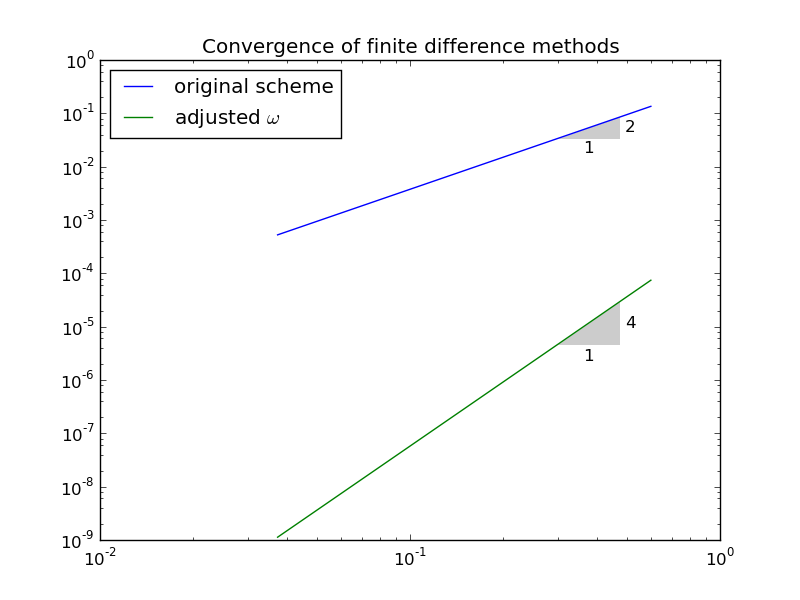## Scaled model

It is advantageous to use dimensionless variables in simulations, because fewer parameters need to be set. The present problem is made dimensionless by introducing dimensionless variables $$\bar t = t/t_c$$ and $$\bar u = u/u_c$$, where $$t_c$$ and $$u_c$$ are characteristic scales for $$t$$ and $$u$$, respectively. We refer to the section Undamped vibrations without forcing in the book Scaling of differential equations  for all details about this scaling.

The scaled ODE problem reads $$\frac{u_c}{t_c^2}\frac{d^2\bar u}{d\bar t^2} + u_c\bar u = 0,\quad u_c\bar u(0) = I,\ \frac{u_c}{t_c}\frac{d\bar u}{d\bar t}(0)=0\tp$$ A common choice is to take $$t_c$$ as one period of the oscillations, $$t_c = 2\pi/w$$, and $$u_c=I$$. This gives the dimensionless model $$\begin{equation} \frac{d^2\bar u}{d\bar t^2} + 4\pi^2 \bar u = 0,\quad \bar u(0)=1,\ \bar u^{\prime}(0)=0\tp \tag{1.13} \end{equation}$$ Observe that there are no physical parameters in (1.13)! We can therefore perform a single numerical simulation $$\bar u(\bar t)$$ and afterwards recover any $$u(t; \omega, I)$$ by $$u(t;\omega, I) = u_c\bar u(t/t_c) = I\bar u(\omega t/(2\pi))\tp$$

We can easily check this assertion: the solution of the scaled problem is $$\bar u(\bar t) = \cos(2\pi\bar t)$$. The formula for $$u$$ in terms of $$\bar u$$ gives $$u = I\cos(\omega t)$$, which is nothing but the solution of the original problem with dimensions.

The scaled model can by run by calling solver(I=1, w=2*pi, dt, T). Each period is now 1 and T simply counts the number of periods. Choosing dt as 1./M gives M time steps per period.

# Visualization of long time simulations

Figure 2 shows a comparison of the exact and numerical solution for the scaled model (1.13) with $$\Delta t=0.1, 0.05$$. From the plot we make the following observations:

• The numerical solution seems to have correct amplitude.
• There is an angular frequency error which is reduced by decreasing the time step.
• The total angular frequency error grows with time.
By angular frequency error we mean that the numerical angular frequency differs from the exact $$\omega$$. This is evident by looking at the peaks of the numerical solution: these have incorrect positions compared with the peaks of the exact cosine solution. The effect can be mathematically expressed by writing the numerical solution as $$I\cos\tilde\omega t$$, where $$\tilde\omega$$ is not exactly equal to $$\omega$$. Later, we shall mathematically quantify this numerical angular frequency $$\tilde\omega$$.

Figure 2: Effect of halving the time step.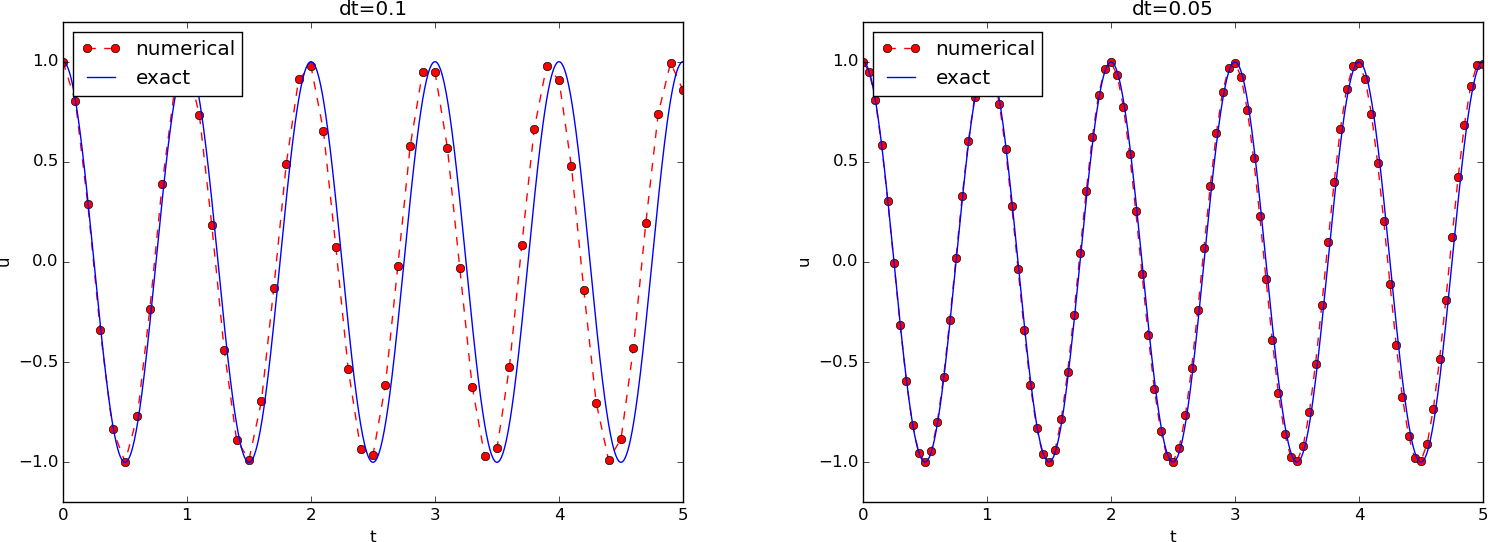## Using a moving plot window

In vibration problems it is often of interest to investigate the system's behavior over long time intervals. Errors in the angular frequency accumulate and become more visible as time grows. We can investigate long time series by introducing a moving plot window that can move along with the $$p$$ most recently computed periods of the solution. The SciTools package contains a convenient tool for this: MovingPlotWindow. Typing pydoc scitools.MovingPlotWindow shows a demo and a description of its use. The function below utilizes the moving plot window and is in fact called by the main function in the vib_undamped module if the number of periods in the simulation exceeds 10.

def visualize_front(u, t, I, w, savefig=False, skip_frames=1):
"""
Visualize u and the exact solution vs t, using a
moving plot window and continuous drawing of the
curves as they evolve in time.
Makes it easy to plot very long time series.
Plots are saved to files if savefig is True.
Only each skip_frames-th plot is saved (e.g., if
skip_frame=10, only each 10th plot is saved to file;
this is convenient if plot files corresponding to
different time steps are to be compared).
"""
import scitools.std as st
from scitools.MovingPlotWindow import MovingPlotWindow
from math import pi

# Remove all old plot files tmp_*.png
import glob, os
for filename in glob.glob('tmp_*.png'):
os.remove(filename)

P = 2*pi/w  # one period
umin = 1.2*u.min();  umax = -umin
dt = t - t
plot_manager = MovingPlotWindow(
window_width=8*P,
dt=dt,
yaxis=[umin, umax],
mode='continuous drawing')
frame_counter = 0
for n in range(1,len(u)):
if plot_manager.plot(n):
s = plot_manager.first_index_in_plot
st.plot(t[s:n+1], u[s:n+1], 'r-1',
t[s:n+1], I*cos(w*t)[s:n+1], 'b-1',
title='t=%6.3f' % t[n],
axis=plot_manager.axis(),
show=not savefig) # drop window if savefig
if savefig and n % skip_frames == 0:
filename = 'tmp_%04d.png' % frame_counter
st.savefig(filename)
print 'making plot file', filename, 'at t=%g' % t[n]
frame_counter += 1
plot_manager.update(n)


We run the scaled problem (the default values for the command-line arguments --I and --w correspond to the scaled problem) for 40 periods with 20 time steps per period:

Terminal> python vib_undamped.py --dt 0.05 --num_periods 40


The moving plot window is invoked, and we can follow the numerical and exact solutions as time progresses. From this demo we see that the angular frequency error is small in the beginning, and that it becomes more prominent with time. A new run with $$\Delta t=0.1$$ (i.e., only 10 time steps per period) clearly shows that the phase errors become significant even earlier in the time series, deteriorating the solution further.

## Making animations

### Producing standard video formats

The visualize_front function stores all the plots in files whose names are numbered: tmp_0000.png, tmp_0001.png, tmp_0002.png, and so on. From these files we may make a movie. The Flash format is popular,

Terminal> ffmpeg -r 25 -i tmp_%04d.png -c:v flv movie.flv


The ffmpeg program can be replaced by the avconv program in the above command if desired (but at the time of this writing it seems to be more momentum in the ffmpeg project). The -r option should come first and describes the number of frames per second in the movie (even if we would like to have slow movies, keep this number as large as 25, otherwise files are skipped from the movie). The -i option describes the name of the plot files. Other formats can be generated by changing the video codec and equipping the video file with the right extension:

 Format Codec and filename Flash -c:v flv movie.flv MP4 -c:v libx264 movie.mp4 WebM -c:v libvpx movie.webm Ogg -c:v libtheora movie.ogg

The video file can be played by some video player like vlc, mplayer, gxine, or totem, e.g.,

Terminal> vlc movie.webm


A web page can also be used to play the movie. Today's standard is to use the HTML5 video tag:

<video autoplay loop controls
<source src='movie.webm'  type='video/webm; codecs="vp8, vorbis"'>
</video>


Modern browsers do not support all of the video formats. MP4 is needed to successfully play the videos on Apple devices that use the Safari browser. WebM is the preferred format for Chrome, Opera, Firefox, and Internet Explorer v9+. Flash was a popular format, but older browsers that required Flash can play MP4. All browsers that work with Ogg can also work with WebM. This means that to have a video work in all browsers, the video should be available in the MP4 and WebM formats. The proper HTML code reads

<video autoplay loop controls
<source src='movie.mp4'   type='video/mp4;
codecs="avc1.42E01E, mp4a.40.2"'>
<source src='movie.webm'  type='video/webm;
codecs="vp8, vorbis"'>
</video>


The MP4 format should appear first to ensure that Apple devices will load the video correctly.

Caution: number the plot files correctly. To ensure that the individual plot frames are shown in correct order, it is important to number the files with zero-padded numbers (0000, 0001, 0002, etc.). The printf format %04d specifies an integer in a field of width 4, padded with zeros from the left. A simple Unix wildcard file specification like tmp_*.png will then list the frames in the right order. If the numbers in the filenames were not zero-padded, the frame tmp_11.png would appear before tmp_2.png in the movie.

### Playing PNG files in a web browser

The scitools movie command can create a movie player for a set of PNG files such that a web browser can be used to watch the movie. This interface has the advantage that the speed of the movie can easily be controlled, a feature that scientists often appreciate. The command for creating an HTML with a player for a set of PNG files tmp_*.png goes like

Terminal> scitools movie output_file=vib.html fps=4 tmp_*.png


The fps argument controls the speed of the movie ("frames per second").

To watch the movie, load the video file vib.html into some browser, e.g.,

Terminal> google-chrome vib.html  # invoke web page


Clicking on Start movie to see the result. Moving this movie to some other place requires moving vib.html and all the PNG files tmp_*.png:

Terminal> mkdir vib_dt0.1
Terminal> mv tmp_*.png vib_dt0.1
Terminal> mv vib.html vib_dt0.1/index.html


### Making animated GIF files

The convert program from the ImageMagick software suite can be used to produce animated GIF files from a set of PNG files:

Terminal> convert -delay 25 tmp_vib*.png tmp_vib.gif


The -delay option needs an argument of the delay between each frame, measured in 1/100 s, so 4 frames/s here gives 25/100 s delay. Note, however, that in this particular example with $$\Delta t=0.05$$ and 40 periods, making an animated GIF file out of the large number of PNG files is a very heavy process and not considered feasible. Animated GIFs are best suited for animations with not so many frames and where you want to see each frame and play them slowly.

## Using Bokeh to compare graphs

Instead of a moving plot frame, one can use tools that allow panning by the mouse. For example, we can show four periods of several signals in several plots and then scroll with the mouse through the rest of the simulation simultaneously in all the plot windows. The Bokeh plotting library offers such tools, but the plots must be displayed in a web browser. The documentation of Bokeh is excellent, so here we just show how the library can be used to compare a set of $$u$$ curves corresponding to long time simulations. (By the way, the guidance to correct pronunciation of Bokeh in the documentation and on Wikipedia is not directly compatible with a YouTube video...).

Imagine we have performed experiments for a set of $$\Delta t$$ values. We want each curve, together with the exact solution, to appear in a plot, and then arrange all plots in a grid-like fashion: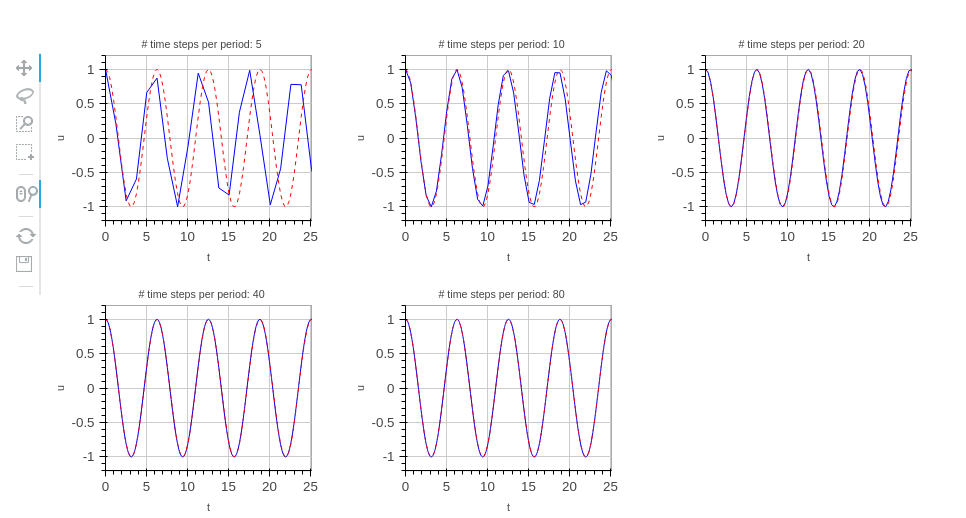Furthermore, we want the axes to couple such that if we move into the future in one plot, all the other plots follows (note the displaced $$t$$ axes!):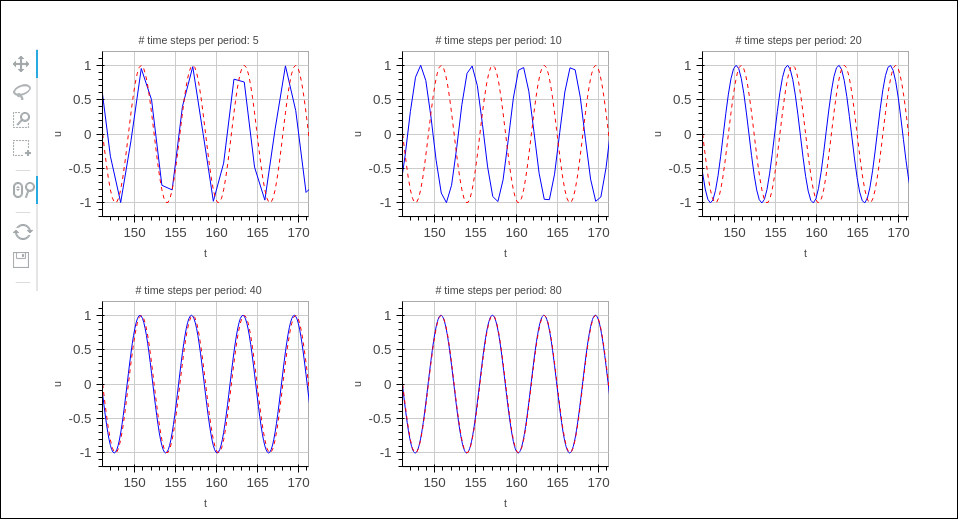Here in HTML format we can include the interactive Bokeh plot. Use the mouse inside one of the plots to scroll into the future!

Figure 3: Interactive Bokeh plot.

A function for creating a Bokeh plot, given a list of u arrays and corresponding t arrays, is implemented below. The code combines data fro different simulations, described compactly in a list of strings legends.

def bokeh_plot(u, t, legends, I, w, t_range, filename):
"""
Make plots for u vs t using the Bokeh library.
u and t are lists (several experiments can be compared).
legens contain legend strings for the various u,t pairs.
"""
if not isinstance(u, (list,tuple)):
u = [u]  # wrap in list
if not isinstance(t, (list,tuple)):
t = [t]  # wrap in list
if not isinstance(legends, (list,tuple)):
legends = [legends]  # wrap in list

import bokeh.plotting as plt
plt.output_file(filename, mode='cdn', title='Comparison')
# Assume that all t arrays have the same range
t_fine = np.linspace(0, t[-1], 1001)  # fine mesh for u_e
tools = 'pan,wheel_zoom,box_zoom,reset,'\
'save,box_select,lasso_select'
u_range = [-1.2*I, 1.2*I]
font_size = '8pt'
p = []  # list of plot objects
# Make the first figure
p_ = plt.figure(
width=300, plot_height=250, title=legends,
x_axis_label='t', y_axis_label='u',
x_range=t_range, y_range=u_range, tools=tools,
title_text_font_size=font_size)
p_.xaxis.axis_label_text_font_size=font_size
p_.yaxis.axis_label_text_font_size=font_size
p_.line(t, u, line_color='blue')
u_e = u_exact(t_fine, I, w)
p_.line(t_fine, u_e, line_color='red', line_dash='4 4')
p.append(p_)
# Make the rest of the figures and attach their axes to
# the first figure's axes
for i in range(1, len(t)):
p_ = plt.figure(
width=300, plot_height=250, title=legends[i],
x_axis_label='t', y_axis_label='u',
x_range=p.x_range, y_range=p.y_range, tools=tools,
title_text_font_size=font_size)
p_.xaxis.axis_label_text_font_size = font_size
p_.yaxis.axis_label_text_font_size = font_size
p_.line(t[i], u[i], line_color='blue')
p_.line(t_fine, u_e, line_color='red', line_dash='4 4')
p.append(p_)

# Arrange all plots in a grid with 3 plots per row
grid = [[]]
for i, p_ in enumerate(p):
grid[-1].append(p_)
if (i+1) % 3 == 0:
# New row
grid.append([])
plot = plt.gridplot(grid, toolbar_location='left')
plt.save(plot)
plt.show(plot)


A particular example using the bokeh_plot function appears below.

def demo_bokeh():
"""Solve a scaled ODE u'' + u = 0."""
from math import pi
w = 1.0        # Scaled problem (frequency)
P = 2*np.pi/w  # Period
num_steps_per_period = [5, 10, 20, 40, 80]
T = 40*P       # Simulation time: 40 periods
u = []         # List of numerical solutions
t = []         # List of corresponding meshes
legends = []
for n in num_steps_per_period:
dt = P/n
u_, t_ = solver(I=1, w=w, dt=dt, T=T)
u.append(u_)
t.append(t_)
legends.append('# time steps per period: %d' % n)
bokeh_plot(u, t, legends, I=1, w=w, t_range=[0, 4*P],
filename='tmp.html')


## Using a line-by-line ascii plotter

Plotting functions vertically, line by line, in the terminal window using ascii characters only is a simple, fast, and convenient visualization technique for long time series. Note that the time axis then is positive downwards on the screen, so we can let the solution be visualized "forever". The tool scitools.avplotter.Plotter makes it easy to create such plots:

def visualize_front_ascii(u, t, I, w, fps=10):
"""
Plot u and the exact solution vs t line by line in a
terminal window (only using ascii characters).
Makes it easy to plot very long time series.
"""
from scitools.avplotter import Plotter
import time
from math import pi
P = 2*pi/w
umin = 1.2*u.min();  umax = -umin

p = Plotter(ymin=umin, ymax=umax, width=60, symbols='+o')
for n in range(len(u)):
print p.plot(t[n], u[n], I*cos(w*t[n])), \
'%.1f' % (t[n]/P)
time.sleep(1/float(fps))


The call p.plot returns a line of text, with the $$t$$ axis marked and a symbol + for the first function (u) and o for the second function (the exact solution). Here we append to this text a time counter reflecting how many periods the current time point corresponds to. A typical output ($$\omega =2\pi$$, $$\Delta t=0.05$$) looks like this:

                              |                       o+      14.0
|                      + o      14.0
|                  +    o       14.1
|             +     o           14.1
|     +        o                14.2
+|       o                       14.2
+        |                               14.2
+       o       |                               14.3
+     o              |                               14.4
+   o                   |                               14.4
+o                       |                               14.5
o +                      |                               14.5
o    +                  |                               14.6
o      +            |                               14.6
o        +     |                               14.7
o       | +                             14.7
|        +                      14.8
|       o       +               14.8
|              o     +          14.9
|                   o   +       14.9
|                       o+      15.0


## Empirical analysis of the solution

For oscillating functions like those in Figure 2 we may compute the amplitude and frequency (or period) empirically. That is, we run through the discrete solution points $$(t_n, u_n)$$ and find all maxima and minima points. The distance between two consecutive maxima (or minima) points can be used as estimate of the local period, while half the difference between the $$u$$ value at a maximum and a nearby minimum gives an estimate of the local amplitude.

The local maxima are the points where $$\begin{equation} u^{n-1} < u^n > u^{n+1},\quad n=1,\ldots,N_t-1, \tag{1.14} \end{equation}$$ and the local minima are recognized by $$\begin{equation} u^{n-1} > u^n < u^{n+1},\quad n=1,\ldots,N_t-1 \tp \tag{1.15} \end{equation}$$ In computer code this becomes

def minmax(t, u):
minima = []; maxima = []
for n in range(1, len(u)-1, 1):
if u[n-1] > u[n] < u[n+1]:
minima.append((t[n], u[n]))
if u[n-1] < u[n] > u[n+1]:
maxima.append((t[n], u[n]))
return minima, maxima


Note that the two returned objects are lists of tuples.

Let $$(t_i, e_i)$$, $$i=0,\ldots,M-1$$, be the sequence of all the $$M$$ maxima points, where $$t_i$$ is the time value and $$e_i$$ the corresponding $$u$$ value. The local period can be defined as $$p_i=t_{i+1}-t_i$$. With Python syntax this reads

def periods(maxima):
p = [extrema[n] - maxima[n-1]
for n in range(1, len(maxima))]
return np.array(p)


The list p created by a list comprehension is converted to an array since we probably want to compute with it, e.g., find the corresponding frequencies 2*pi/p.

Having the minima and the maxima, the local amplitude can be calculated as the difference between two neighboring minimum and maximum points:

def amplitudes(minima, maxima):
a = [(abs(maxima[n] - minima[n]))/2.0
for n in range(min(len(minima),len(maxima)))]
return np.array(a)


The code segments are found in the file vib_empirical_analysis.py.

Since a[i] and p[i] correspond to the $$i$$-th amplitude estimate and the $$i$$-th period estimate, respectively, it is most convenient to visualize the a and p values with the index i on the horizontal axis. (There is no unique time point associated with either of these estimate since values at two different time points were used in the computations.)

In the analysis of very long time series, it is advantageous to compute and plot p and a instead of $$u$$ to get an impression of the development of the oscillations. Let us do this for the scaled problem and $$\Delta t=0.1, 0.05, 0.01$$. A ready-made function

plot_empirical_freq_and_amplitude(u, t, I, w)


computes the empirical amplitudes and periods, and creates a plot where the amplitudes and angular frequencies are visualized together with the exact amplitude I and the exact angular frequency w. We can make a little program for creating the plot:

from vib_undamped import solver, plot_empirical_freq_and_amplitude
from math import pi
dt_values = [0.1, 0.05, 0.01]
u_cases = []
t_cases = []
for dt in dt_values:
# Simulate scaled problem for 40 periods
u, t = solver(I=1, w=2*pi, dt=dt, T=40)
u_cases.append(u)
t_cases.append(t)
plot_empirical_freq_and_amplitude(u_cases, t_cases, I=1, w=2*pi)


Figure 4 shows the result: we clearly see that lowering $$\Delta t$$ improves the angular frequency significantly, while the amplitude seems to be more accurate. The lines with $$\Delta t=0.01$$, corresponding to 100 steps per period, can hardly be distinguished from the exact values. The next section shows how we can get mathematical insight into why amplitudes are good while frequencies are more inaccurate.

Figure 4: Empirical angular frequency (left) and amplitude (right) for three different time steps.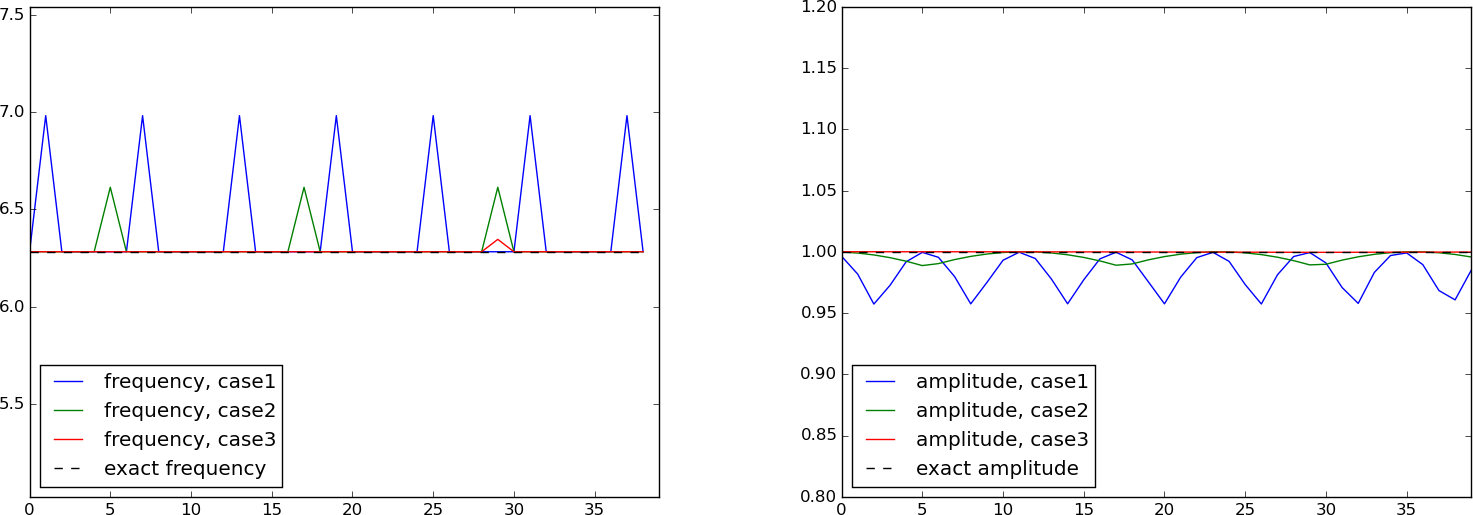# Analysis of the numerical scheme

## Deriving a solution of the numerical scheme

After having seen the phase error grow with time in the previous section, we shall now quantify this error through mathematical analysis. The key tool in the analysis will be to establish an exact solution of the discrete equations. The difference equation (1.7) has constant coefficients and is homogeneous. Such equations are known to have solutions on the form $$u^n=CA^n$$, where $$A$$ is some number to be determined from the difference equation and $$C$$ is found as the initial condition ($$C=I$$). Recall that $$n$$ in $$u^n$$ is a superscript labeling the time level, while $$n$$ in $$A^n$$ is an exponent.

With oscillating functions as solutions, the algebra will be considerably simplified if we seek an $$A$$ on the form $$A=e^{i\tilde\omega \Delta t},$$ and solve for the numerical frequency $$\tilde\omega$$ rather than $$A$$. Note that $$i=\sqrt{-1}$$ is the imaginary unit. (Using a complex exponential function gives simpler arithmetics than working with a sine or cosine function.) We have $$A^n = e^{i\tilde\omega \Delta t\, n}=e^{i\tilde\omega t_n} = \cos (\tilde\omega t_n) + i\sin(\tilde \omega t_n) \tp$$ The physically relevant numerical solution can be taken as the real part of this complex expression.

The calculations go as \begin{align*} [D_tD_t u]^n &= \frac{u^{n+1} - 2u^n + u^{n-1}}{\Delta t^2}\\ &= I\frac{A^{n+1} - 2A^n + A^{n-1}}{\Delta t^2}\\ &= \frac{I}{\Delta t^{2}}(e^{i\tilde\omega(t_n+\Delta t)} - 2e^{i\tilde\omega t_n} + e^{i\tilde\omega(t_n-\Delta t)})\\ &= Ie^{i\tilde\omega t_n}\frac{1}{\Delta t^2}\left(e^{i\tilde\omega\Delta t} + e^{i\tilde\omega(-\Delta t)} - 2\right)\\ &= Ie^{i\tilde\omega t_n}\frac{2}{\Delta t^2}\left(\cosh(i\tilde\omega\Delta t) -1 \right)\\ &= Ie^{i\tilde\omega t_n}\frac{2}{\Delta t^2}\left(\cos(\tilde\omega\Delta t) -1 \right)\\ &= -Ie^{i\tilde\omega t_n}\frac{4}{\Delta t^2}\sin^2(\frac{\tilde\omega\Delta t}{2}) \end{align*} The last line follows from the relation $$\cos x - 1 = -2\sin^2(x/2)$$ (try cos(x)-1 in wolframalpha.com to see the formula).

The scheme (1.7) with $$u^n=Ie^{i\tilde\omega\Delta t\, n}$$ inserted now gives $$\begin{equation} -Ie^{i\tilde\omega t_n} \frac{4}{\Delta t^2}\sin^2(\frac{\tilde\omega\Delta t}{2}) + \omega^2 Ie^{i\tilde\omega t_n} = 0, \tag{1.16} \end{equation}$$ which after dividing by $$Ie^{i\tilde\omega t_n}$$ results in $$\begin{equation} \frac{4}{\Delta t^2}\sin^2(\frac{\tilde\omega\Delta t}{2}) = \omega^2 \tp \tag{1.17} \end{equation}$$ The first step in solving for the unknown $$\tilde\omega$$ is $$\sin^2(\frac{\tilde\omega\Delta t}{2}) = \left(\frac{\omega\Delta t}{2}\right)^2 \tp$$ Then, taking the square root, applying the inverse sine function, and multiplying by $$2/\Delta t$$, results in $$\begin{equation} \tilde\omega = \pm \frac{2}{\Delta t}\sin^{-1}\left(\frac{\omega\Delta t}{2}\right) \tp \tag{1.18} \end{equation}$$

## The error in the numerical frequency

The first observation of (1.18) tells that there is a phase error since the numerical frequency $$\tilde\omega$$ never equals the exact frequency $$\omega$$. But how good is the approximation (1.18)? That is, what is the error $$\omega - \tilde\omega$$ or $$\tilde\omega/\omega$$? Taylor series expansion for small $$\Delta t$$ may give an expression that is easier to understand than the complicated function in (1.18):

>>> from sympy import *
>>> dt, w = symbols('dt w')
>>> w_tilde_e = 2/dt*asin(w*dt/2)
>>> w_tilde_series = w_tilde_e.series(dt, 0, 4)
>>> print w_tilde_series
w + dt**2*w**3/24 + O(dt**4)


This means that

$$\begin{equation} \tilde\omega = \omega\left( 1 + \frac{1}{24}\omega^2\Delta t^2\right) + \Oof{\Delta t^4} \tp \tag{1.19} \end{equation}$$ The error in the numerical frequency is of second-order in $$\Delta t$$, and the error vanishes as $$\Delta t\rightarrow 0$$. We see that $$\tilde\omega > \omega$$ since the term $$\omega^3\Delta t^2/24 >0$$ and this is by far the biggest term in the series expansion for small $$\omega\Delta t$$. A numerical frequency that is too large gives an oscillating curve that oscillates too fast and therefore "lags behind" the exact oscillations, a feature that can be seen in the left plot in Figure 2.

Figure 5 plots the discrete frequency (1.18) and its approximation (1.19) for $$\omega =1$$ (based on the program vib_plot_freq.py). Although $$\tilde\omega$$ is a function of $$\Delta t$$ in (1.19), it is misleading to think of $$\Delta t$$ as the important discretization parameter. It is the product $$\omega\Delta t$$ that is the key discretization parameter. This quantity reflects the number of time steps per period of the oscillations. To see this, we set $$P=N_P\Delta t$$, where $$P$$ is the length of a period, and $$N_P$$ is the number of time steps during a period. Since $$P$$ and $$\omega$$ are related by $$P=2\pi/\omega$$, we get that $$\omega\Delta t = 2\pi/N_P$$, which shows that $$\omega\Delta t$$ is directly related to $$N_P$$.

The plot shows that at least $$N_P\sim 25-30$$ points per period are necessary for reasonable accuracy, but this depends on the length of the simulation ($$T$$) as the total phase error due to the frequency error grows linearly with time (see Exercise 1.2: Show linear growth of the phase with time).

Figure 5: Exact discrete frequency and its second-order series expansion.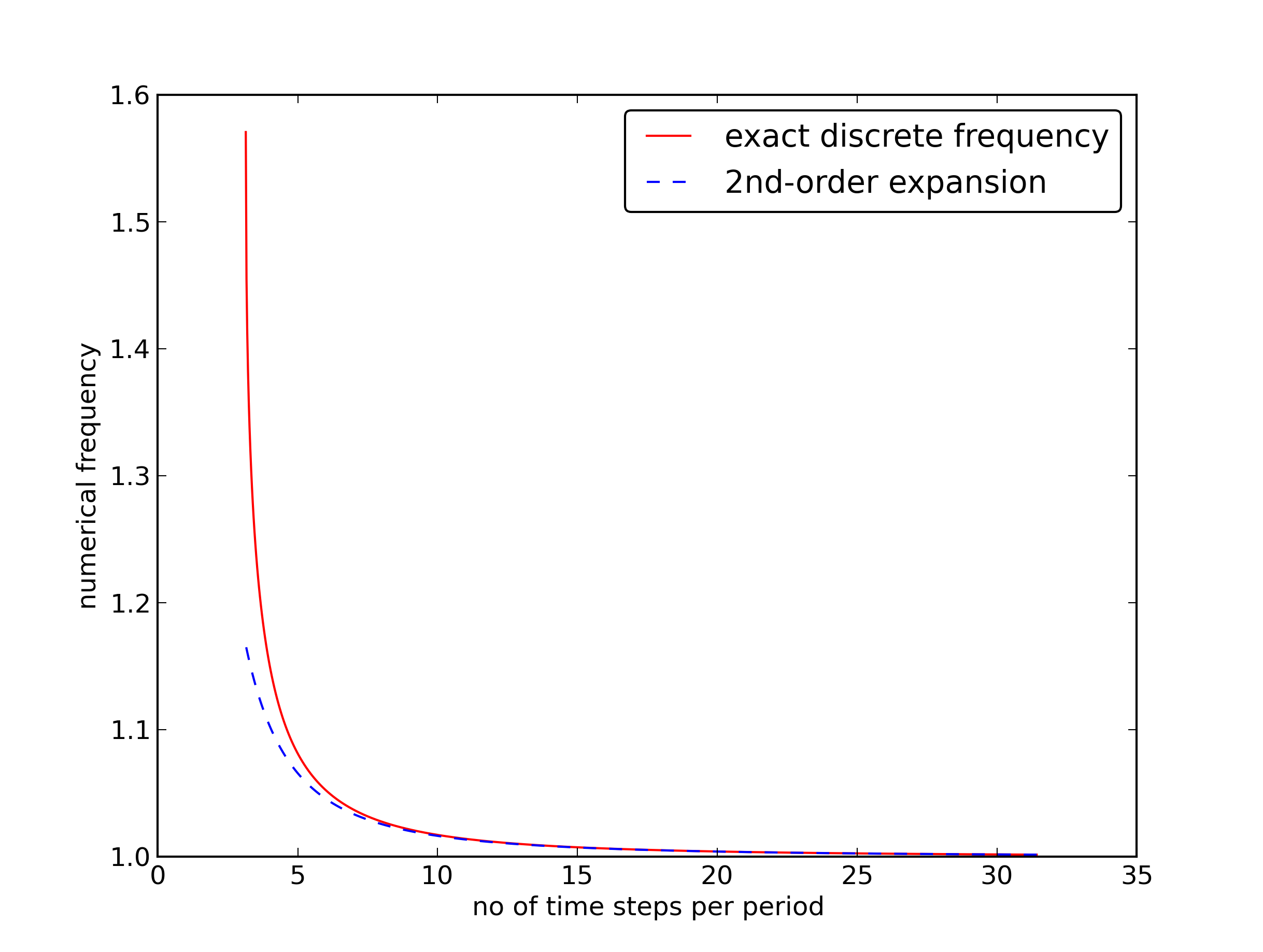## Empirical convergence rates and adjusted $$\omega$$

The expression (1.19) suggest that adjusting omega to $$\omega\left( 1 - \frac{1}{24}\omega^2\Delta t^2\right),$$ could have effect on the convergence rate of the global error in $$u$$ (cf. the section Verification). With the convergence_rates function in vib_undamped.py we can easily check this. A special solver, with adjusted $$w$$, is available as the function solver_adjust_w. A call to convergence_rates with this solver reveals that the rate is 4.0! With the original, physical $$\omega$$ the rate is 2.0 - as expected from using second-order finite difference approximations, as expected from the forthcoming derivation of the global error, and as expected from truncation error analysis analysis as explained in Linear model without damping.

Adjusting $$\omega$$ is an ideal trick for this simple problem, but when adding damping and nonlinear terms, we have no simple formula for the impact on $$\omega$$, and therefore we cannot use the trick.

## Exact discrete solution

Perhaps more important than the $$\tilde\omega = \omega + {\cal O}(\Delta t^2)$$ result found above is the fact that we have an exact discrete solution of the problem: $$\begin{equation} u^n = I\cos\left(\tilde\omega n\Delta t\right),\quad \tilde\omega = \frac{2}{\Delta t}\sin^{-1}\left(\frac{\omega\Delta t}{2}\right) \tp \tag{1.20} \end{equation}$$ We can then compute the error mesh function $$\begin{equation} e^n = \uex(t_n) - u^n = I\cos\left(\omega n\Delta t\right) - I\cos\left(\tilde\omega n\Delta t\right)\tp \tag{1.21} \end{equation}$$ From the formula $$\cos 2x - \cos 2y = -2\sin(x-y)\sin(x+y)$$ we can rewrite $$e^n$$ so the expression is easier to interpret: $$\begin{equation} e^n = -2I\sin\left(t\half\left( \omega - \tilde\omega\right)\right) \sin\left(t\half\left( \omega + \tilde\omega\right)\right)\tp \tag{1.22} \end{equation}$$

The error mesh function is ideal for verification purposes and you are strongly encouraged to make a test based on (1.20) by doing Exercise 1.11: Use an exact discrete solution for verification.

## Convergence

We can use (1.19), (1.21), or (1.22) to show convergence of the numerical scheme, i.e., $$e^n\rightarrow 0$$ as $$\Delta t\rightarrow 0$$, which implies that the numerical solution approaches the exact solution as $$\Delta t$$ approaches to zero. We have that $$\lim_{\Delta t\rightarrow 0} \tilde\omega = \lim_{\Delta t\rightarrow 0} \frac{2}{\Delta t}\sin^{-1}\left(\frac{\omega\Delta t}{2}\right) = \omega,$$ by L'Hopital's rule. This result could also been computed WolframAlpha, or we could use the limit functionality in sympy:

>>> import sympy as sym
>>> dt, w = sym.symbols('x w')
>>> sym.limit((2/dt)*sym.asin(w*dt/2), dt, 0, dir='+')
w


Also (1.19) can be used to establish that $$\tilde\omega\rightarrow\omega$$ when $$\Delta t\rightarrow 0$$. It then follows from the expression(s) for $$e^n$$ that $$e^n\rightarrow 0$$.

## The global error

To achieve more analytical insight into the nature of the global error, we can Taylor expand the error mesh function (1.21). Since $$\tilde\omega$$ in (1.18) contains $$\Delta t$$ in the denominator we use the series expansion for $$\tilde\omega$$ inside the cosine function. A relevant sympy session is

>>> from sympy import *
>>> dt, w, t = symbols('dt w t')
>>> w_tilde_e = 2/dt*asin(w*dt/2)
>>> w_tilde_series = w_tilde_e.series(dt, 0, 4)
>>> w_tilde_series
w + dt**2*w**3/24 + O(dt**4)


Series expansions in sympy have the inconvenient O() term that prevents further calculations with the series. We can use the removeO() command to get rid of the O() term:

>>> w_tilde_series = w_tilde_series.removeO()
>>> w_tilde_series
dt**2*w**3/24 + w


Using this w_tilde_series expression for $$\tilde w$$ in (1.21), dropping $$I$$ (which is a common factor), and performing a series expansion of the error yields

>>> error = cos(w*t) - cos(w_tilde_series*t)
>>> error.series(dt, 0, 6)
dt**2*t*w**3*sin(t*w)/24 + dt**4*t**2*w**6*cos(t*w)/1152 + O(dt**6)


Since we are mainly interested in the leading-order term in such expansions (the term with lowest power in $$\Delta t$$, which goes most slowly to zero), we use the .as_leading_term(dt) construction to pick out this term:

>>> error.series(dt, 0, 6).as_leading_term(dt)
dt**2*t*w**3*sin(t*w)/24


The last result means that the leading order global (true) error at a point $$t$$ is proportional to $$\omega^3t\Delta t^2$$. Considering only the discrete $$t_n$$ values for $$t$$, $$t_n$$ is related to $$\Delta t$$ through $$t_n=n\Delta t$$. The factor $$\sin(\omega t)$$ can at most be 1, so we use this value to bound the leading-order expression to its maximum value $$e^n = \frac{1}{24}n\omega^3\Delta t^3\tp$$ This is the dominating term of the error at a point.

We are interested in the accumulated global error, which can be taken as the $$\ell^2$$ norm of $$e^n$$. The norm is simply computed by summing contributions from all mesh points: $$||e^n||_{\ell^2}^2 = \Delta t\sum_{n=0}^{N_t} \frac{1}{24^2}n^2\omega^6\Delta t^6 =\frac{1}{24^2}\omega^6\Delta t^7 \sum_{n=0}^{N_t} n^2\tp$$ The sum $$\sum_{n=0}^{N_t} n^2$$ is approximately equal to $$\frac{1}{3}N_t^3$$. Replacing $$N_t$$ by $$T/\Delta t$$ and taking the square root gives the expression $$||e^n||_{\ell^2} = \frac{1}{24}\sqrt{\frac{T^3}{3}}\omega^3\Delta t^2\tp$$ This is our expression for the global (or integrated) error. A primary result from this expression is that the global error is proportional to $$\Delta t^2$$.

## Stability

Looking at (1.20), it appears that the numerical solution has constant and correct amplitude, but an error in the angular frequency. A constant amplitude is not necessarily the case, however! To see this, note that if only $$\Delta t$$ is large enough, the magnitude of the argument to $$\sin^{-1}$$ in (1.18) may be larger than 1, i.e., $$\omega\Delta t/2 > 1$$. In this case, $$\sin^{-1}(\omega\Delta t/2)$$ has a complex value and therefore $$\tilde\omega$$ becomes complex. Type, for example, asin(x) in wolframalpha.com to see basic properties of $$\sin^{-1} (x)$$).

A complex $$\tilde\omega$$ can be written $$\tilde\omega = \tilde\omega_r + i\tilde\omega_i$$. Since $$\sin^{-1}(x)$$ has a negative imaginary part for $$x>1$$, $$\tilde\omega_i < 0$$, which means that $$e^{i\tilde\omega t}=e^{-\tilde\omega_i t}e^{i\tilde\omega_r t}$$ will lead to exponential growth in time because $$e^{-\tilde\omega_i t}$$ with $$\tilde\omega_i < 0$$ has a positive exponent.

Stability criterion. We do not tolerate growth in the amplitude since such growth is not present in the exact solution. Therefore, we must impose a stability criterion so that the argument in the inverse sine function leads to real and not complex values of $$\tilde\omega$$. The stability criterion reads $$\begin{equation} \frac{\omega\Delta t}{2} \leq 1\quad\Rightarrow\quad \Delta t \leq \frac{2}{\omega} \tp \tag{1.23} \end{equation}$$

With $$\omega =2\pi$$, $$\Delta t > \pi^{-1} = 0.3183098861837907$$ will give growing solutions. Figure 6 displays what happens when $$\Delta t =0.3184$$, which is slightly above the critical value: $$\Delta t =\pi^{-1} + 9.01\cdot 10^{-5}$$.

Figure 6: Growing, unstable solution because of a time step slightly beyond the stability limit.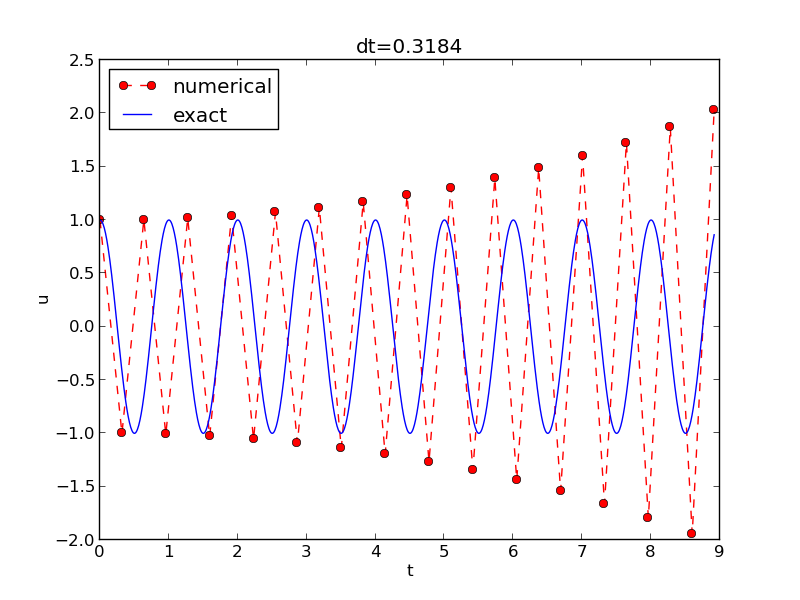## About the accuracy at the stability limit

An interesting question is whether the stability condition $$\Delta t < 2/\omega$$ is unfortunate, or more precisely: would it be meaningful to take larger time steps to speed up computations? The answer is a clear no. At the stability limit, we have that $$\sin^{-1}\omega\Delta t/2 = \sin^{-1} 1 = \pi/2$$, and therefore $$\tilde\omega = \pi/\Delta t$$. (Note that the approximate formula (1.19) is very inaccurate for this value of $$\Delta t$$ as it predicts $$\tilde\omega = 2.34/pi$$, which is a 25 percent reduction.) The corresponding period of the numerical solution is $$\tilde P=2\pi/\tilde\omega = 2\Delta t$$, which means that there is just one time step $$\Delta t$$ between a peak (maximum) and a through (minimum) in the numerical solution. This is the shortest possible wave that can be represented in the mesh! In other words, it is not meaningful to use a larger time step than the stability limit.

Also, the error in angular frequency when $$\Delta t = 2/\omega$$ is severe: Figure 7 shows a comparison of the numerical and analytical solution with $$\omega = 2\pi$$ and $$\Delta t = 2/\omega = \pi^{-1}$$. Already after one period, the numerical solution has a through while the exact solution has a peak (!). The error in frequency when $$\Delta t$$ is at the stability limit becomes $$\omega - \tilde\omega = \omega(1-\pi/2)\approx -0.57\omega$$. The corresponding error in the period is $$P - \tilde P \approx 0.36P$$. The error after $$m$$ periods is then $$0.36mP$$. This error has reached half a period when $$m=1/(2\cdot 0.36)\approx 1.38$$, which theoretically confirms the observations in Figure 7 that the numerical solution is a through ahead of a peak already after one and a half period. Consequently, $$\Delta t$$ should be chosen much less than the stability limit to achieve meaningful numerical computations.

Figure 7: Numerical solution with $$\Delta t$$ exactly at the stability limit.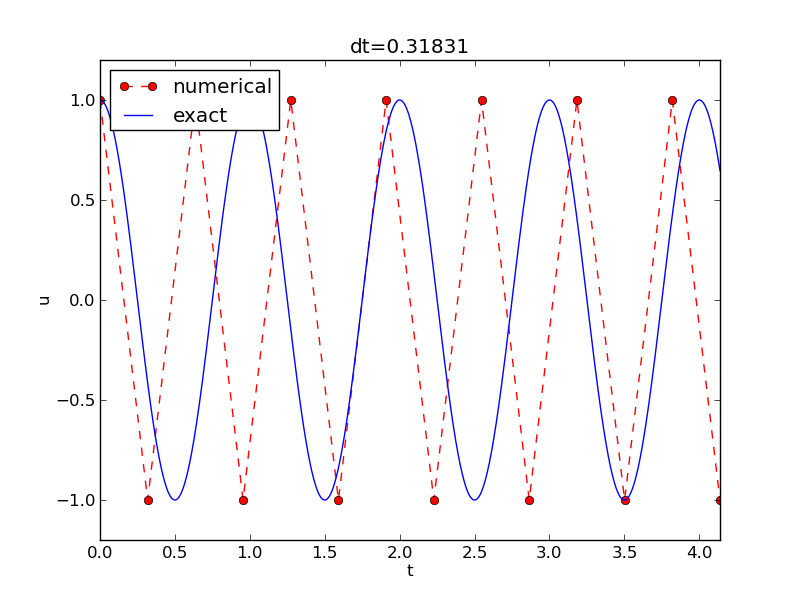Summary. From the accuracy and stability analysis we can draw three important conclusions:
1. The key parameter in the formulas is $$p=\omega\Delta t$$. The period of oscillations is $$P=2\pi/\omega$$, and the number of time steps per period is $$N_P=P/\Delta t$$. Therefore, $$p=\omega\Delta t = 2\pi/N_P$$, showing that the critical parameter is the number of time steps per period. The smallest possible $$N_P$$ is 2, showing that $$p\in (0,\pi]$$.
2. Provided $$p\leq 2$$, the amplitude of the numerical solution is constant.
3. The ratio of the numerical angular frequency and the exact one is $$\tilde\omega/\omega \approx 1 + \frac{1}{24}p^2$$. The error $$\frac{1}{24}p^2$$ leads to wrongly displaced peaks of the numerical solution, and the error in peak location grows linearly with time (see Exercise 1.2: Show linear growth of the phase with time).

# Alternative schemes based on 1st-order equations

A standard technique for solving second-order ODEs is to rewrite them as a system of first-order ODEs and then choose a solution strategy from the vast collection of methods for first-order ODE systems. Given the second-order ODE problem $$u^{\prime\prime} + \omega^2 u = 0,\quad u(0)=I,\ u^{\prime}(0)=0,$$ we introduce the auxiliary variable $$v=u^{\prime}$$ and express the ODE problem in terms of first-order derivatives of $$u$$ and $$v$$: \begin{align} u^{\prime} &= v, \tag{1.24}\\ v^{\prime} &= -\omega^2 u \tag{1.25} \tp \end{align} The initial conditions become $$u(0)=I$$ and $$v(0)=0$$.

## The Forward Euler scheme

A Forward Euler approximation to our $$2\times 2$$ system of ODEs (1.24)-(1.25) becomes \begin{align} \lbrack D_t^+ u &= v\rbrack^n, \tag{1.26}\\ \lbrack D_t^+ v &= -\omega^2 u\rbrack^n, \tag{1.27} \end{align} or written out, \begin{align} u^{n+1} &= u^n + \Delta t v^n, \tag{1.28}\\ v^{n+1} &= v^n -\Delta t \omega^2 u^n \tag{1.29} \tp \end{align}

Let us briefly compare this Forward Euler method with the centered difference scheme for the second-order differential equation. We have from (1.28) and (1.29) applied at levels $$n$$ and $$n-1$$ that $$u^{n+1} = u^n + \Delta t v^n = u^n + \Delta t (v^{n-1} -\Delta t \omega^2 u^{n-1})\tp$$ Since from (1.28) $$v^{n-1} = \frac{1}{\Delta t}(u^{n}-u^{n-1}),$$ it follows that $$u^{n+1} = 2u^n - u^{n-1} -\Delta t^2\omega^2 u^{n-1},$$ which is very close to the centered difference scheme, but the last term is evaluated at $$t_{n-1}$$ instead of $$t_n$$. Rewriting, so that $$\Delta t^2\omega^2u^{n-1}$$ appears alone on the right-hand side, and then dividing by $$\Delta t^2$$, the new left-hand side is an approximation to $$u^{\prime\prime}$$ at $$t_n$$, while the right-hand side is sampled at $$t_{n-1}$$. All terms should be sampled at the same mesh point, so using $$\omega^2 u^{n-1}$$ instead of $$\omega^2 u^n$$ points to a kind of mathematical error in the derivation of the scheme. This error turns out to be rather crucial for the accuracy of the Forward Euler method applied to vibration problems (the section Comparison of schemes has examples).

The reasoning above does not imply that the Forward Euler scheme is not correct, but more that it is almost equivalent to a second-order accurate scheme for the second-order ODE formulation, and that the error committed has to do with a wrong sampling point.

## The Backward Euler scheme

A Backward Euler approximation to the ODE system is equally easy to write up in the operator notation: \begin{align} \lbrack D_t^- u &= v\rbrack^{n+1}, \tag{1.30}\\ \lbrack D_t^- v &= -\omega u\rbrack^{n+1} \tp \tag{1.31} \end{align} This becomes a coupled system for $$u^{n+1}$$ and $$v^{n+1}$$: \begin{align} u^{n+1} - \Delta t v^{n+1} &= u^{n}, \tag{1.32}\\ v^{n+1} + \Delta t \omega^2 u^{n+1} &= v^{n} \tag{1.33} \tp \end{align}

We can compare (1.32)-(1.33) with the centered scheme (1.7) for the second-order differential equation. To this end, we eliminate $$v^{n+1}$$ in (1.32) using (1.33) solved with respect to $$v^{n+1}$$. Thereafter, we eliminate $$v^n$$ using (1.32) solved with respect to $$v^{n+1}$$ and also replacing $$n+1$$ by $$n$$ and $$n$$ by $$n-1$$. The resulting equation involving only $$u^{n+1}$$, $$u^n$$, and $$u^{n-1}$$ can be ordered as $$\frac{u^{n+1}-2u^n+u^{n-1}}{\Delta t^2} = -\omega^2 u^{n+1},$$ which has almost the same form as the centered scheme for the second-order differential equation, but the right-hand side is evaluated at $$u^{n+1}$$ and not $$u^n$$. This inconsistent sampling of terms has a dramatic effect on the numerical solution, as we demonstrate in the section Comparison of schemes.

## The Crank-Nicolson scheme

The Crank-Nicolson scheme takes this form in the operator notation: \begin{align} \lbrack D_t u &= \overline{v}^t\rbrack^{n+\half}, \tag{1.34}\\ \lbrack D_t v &= -\omega^2 \overline{u}^t\rbrack^{n+\half} \tp \tag{1.35} \end{align} Writing the equations out and rearranging terms, shows that this is also a coupled system of two linear equations at each time level: \begin{align} u^{n+1} - \half\Delta t v^{n+1} &= u^{n} + \half\Delta t v^{n}, \tag{1.36}\\ v^{n+1} + \half\Delta t \omega^2 u^{n+1} &= v^{n} - \half\Delta t \omega^2 u^{n} \tp \tag{1.37} \end{align}

We may compare also this scheme to the centered discretization of the second-order ODE. It turns out that the Crank-Nicolson scheme is equivalent to the discretization $$\begin{equation} \frac{u^{n+1} - 2u^n + u^{n-1}}{\Delta t^2} = - \omega^2 \frac{1}{4}(u^{n+1} + 2u^n + u^{n-1}) = -\omega^2 u^{n} + \Oof{\Delta t^2}\tp \tag{1.38} \end{equation}$$ That is, the Crank-Nicolson is equivalent to (1.7) for the second-order ODE, apart from an extra term of size $$\Delta t^2$$, but this is an error of the same order as in the finite difference approximation on the left-hand side of the equation anyway. The fact that the Crank-Nicolson scheme is so close to (1.7) makes it a much better method than the Forward or Backward Euler methods for vibration problems, as will be illustrated in the section Comparison of schemes.

Deriving (1.38) is a bit tricky. We start with rewriting the Crank-Nicolson equations as follows \begin{align} u^{n+1} - u^n &= \frac{1}{2}\Delta t(v^{n+1} + v^n), \tag{1.39}\\ v^{n+1} &= v^n -\frac{1}{2}\Delta t\omega^2 (u^{n+1} + u^n), \tag{1.40} \end{align} and add the latter at the previous time level as well: $$\begin{equation} v^{n} = v^{n-1} -\frac{1}{2}\Delta t\omega^2(u^{n} + u^{n-1}) \tag{1.41} \end{equation}$$ We can also rewrite (1.39) at the previous time level as $$\begin{equation} v^{n} + v^{n-1} = \frac{2}{\Delta t}(u^{n} - u^{n-1})\tp \tag{1.42} \end{equation}$$ Inserting (1.40) for $$v^{n+1}$$ in (1.39) and (1.41) for $$v^{n}$$ in (1.39) yields after some reordering: $$u^{n+1} - u^n = \frac{1}{2}(-\frac{1}{2}\Delta t\omega^2 (u^{n+1} + 2u^n + u^{n-1}) + v^n + v^{n-1})\tp$$ Now, $$v^n + v^{n-1}$$ can be eliminated by means of (1.42). The result becomes $$\begin{equation} u^{n+1} - 2u^n + u^{n-1} = -\Delta t^2\omega^2 \frac{1}{4}(u^{n+1} + 2u^n + u^{n-1})\tp \tag{1.43} \end{equation}$$ It can be shown that $$\frac{1}{4}(u^{n+1} + 2u^n + u^{n-1}) \approx u^n + \Oof{\Delta t^2},$$ meaning that (1.43) is an approximation to the centered scheme (1.7) for the second-order ODE where the sampling error in the term $$\Delta t^2\omega^2 u^n$$ is of the same order as the approximation errors in the finite differences, i.e., $$\Oof{\Delta t^2}$$. The Crank-Nicolson scheme written as (1.43) therefore has consistent sampling of all terms at the same time point $$t_n$$.

## Comparison of schemes

We can easily compare methods like the ones above (and many more!) with the aid of the Odespy package. Below is a sketch of the code.

import odespy
import numpy as np

def f(u, t, w=1):
# v, u numbering for EulerCromer to work well
v, u = u  # u is array of length 2 holding our [v, u]
return [-w**2*u, v]

def run_solvers_and_plot(solvers, timesteps_per_period=20,
num_periods=1, I=1, w=2*np.pi):
P = 2*np.pi/w  # duration of one period
dt = P/timesteps_per_period
Nt = num_periods*timesteps_per_period
T = Nt*dt
t_mesh = np.linspace(0, T, Nt+1)

legends = []
for solver in solvers:
solver.set(f_kwargs={'w': w})
solver.set_initial_condition([0, I])
u, t = solver.solve(t_mesh)


There is quite some more code dealing with plots also, and we refer to the source file vib_undamped_odespy.py for details. Observe that keyword arguments in f(u,t,w=1) can be supplied through a solver parameter f_kwargs (dictionary of additional keyword arguments to f).

Specification of the Forward Euler, Backward Euler, and Crank-Nicolson schemes is done like this:

solvers = [
odespy.ForwardEuler(f),
# Implicit methods must use Newton solver to converge
odespy.BackwardEuler(f, nonlinear_solver='Newton'),
odespy.CrankNicolson(f, nonlinear_solver='Newton'),
]


The vib_undamped_odespy.py program makes two plots of the computed solutions with the various methods in the solvers list: one plot with $$u(t)$$ versus $$t$$, and one phase plane plot where $$v$$ is plotted against $$u$$. That is, the phase plane plot is the curve $$(u(t),v(t))$$ parameterized by $$t$$. Analytically, $$u=I\cos(\omega t)$$ and $$v=u^{\prime}=-\omega I\sin(\omega t)$$. The exact curve $$(u(t),v(t))$$ is therefore an ellipse, which often looks like a circle in a plot if the axes are automatically scaled. The important feature, however, is that the exact curve $$(u(t),v(t))$$ is closed and repeats itself for every period. Not all numerical schemes are capable of doing that, meaning that the amplitude instead shrinks or grows with time.

Figure 8 show the results. Note that Odespy applies the label MidpointImplicit for what we have specified as CrankNicolson in the code (CrankNicolson is just a synonym for class MidpointImplicit in the Odespy code). The Forward Euler scheme in Figure 8 has a pronounced spiral curve, pointing to the fact that the amplitude steadily grows, which is also evident in Figure 9. The Backward Euler scheme has a similar feature, except that the spriral goes inward and the amplitude is significantly damped. The changing amplitude and the spiral form decreases with decreasing time step. The Crank-Nicolson scheme looks much more accurate. In fact, these plots tell that the Forward and Backward Euler schemes are not suitable for solving our ODEs with oscillating solutions.

Figure 8: Comparison of classical schemes in the phase plane for two time step values.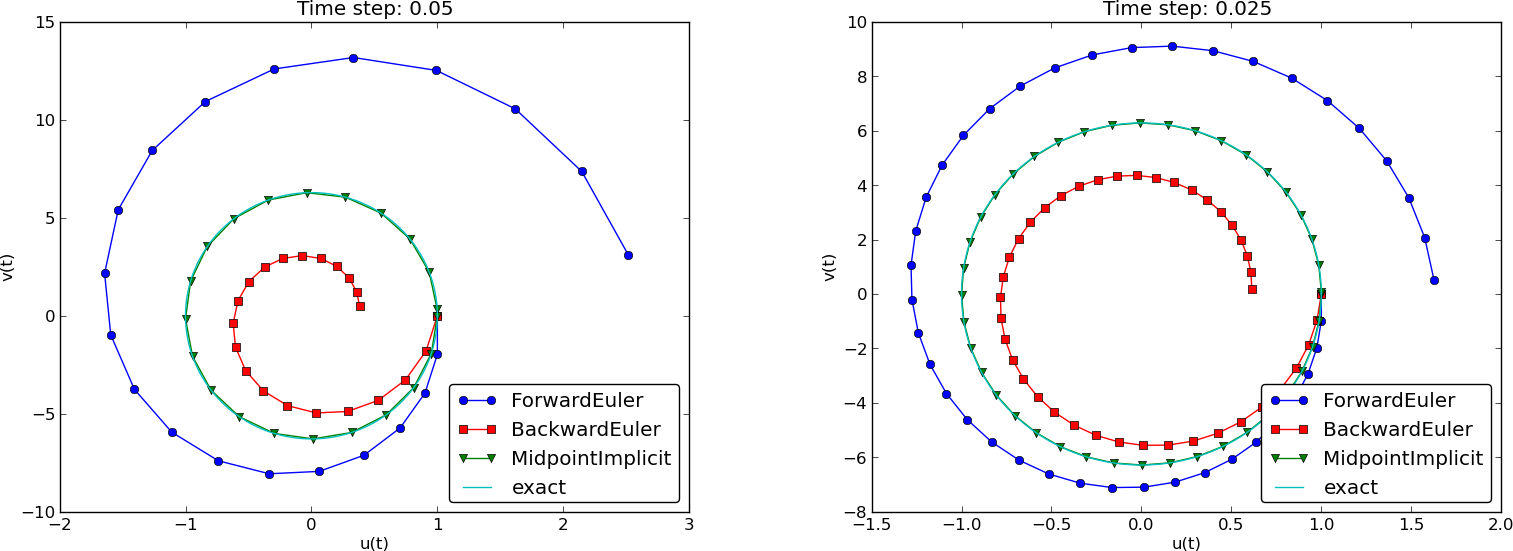Figure 9: Comparison of solution curves for classical schemes.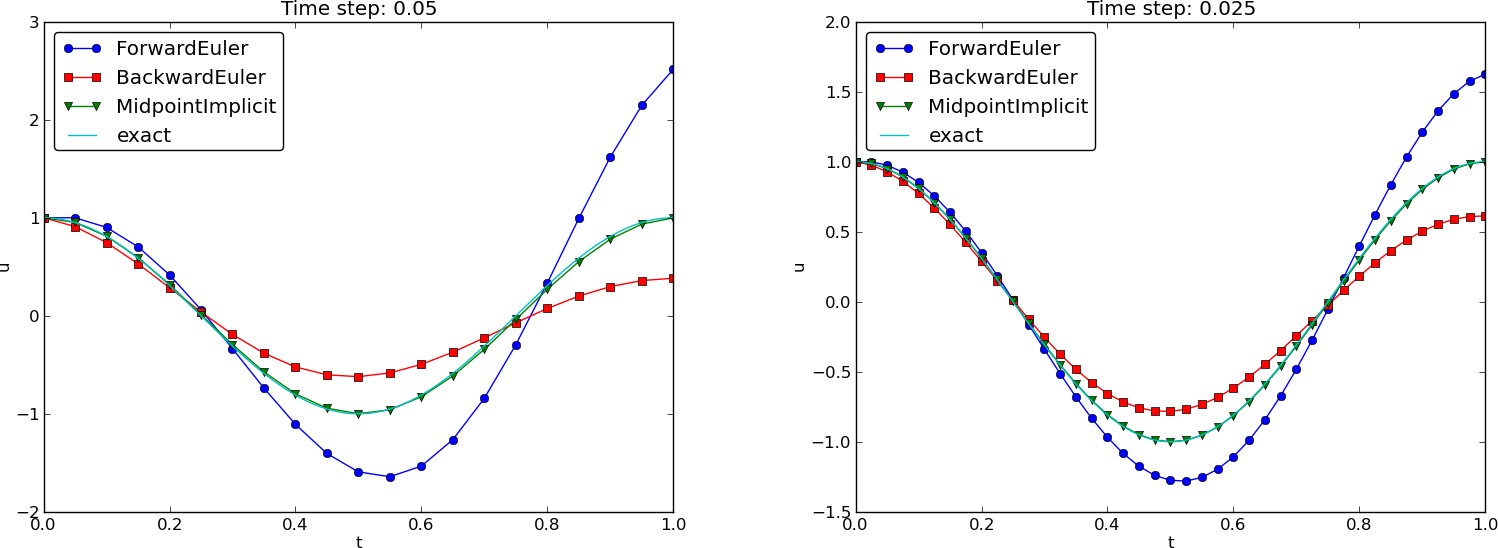## Runge-Kutta methods

We may run two other popular standard methods for first-order ODEs, the 2nd- and 4th-order Runge-Kutta methods, to see how they perform. Figures 10 and 11 show the solutions with larger $$\Delta t$$ values than what was used in the previous two plots.

Figure 10: Comparison of Runge-Kutta schemes in the phase plane.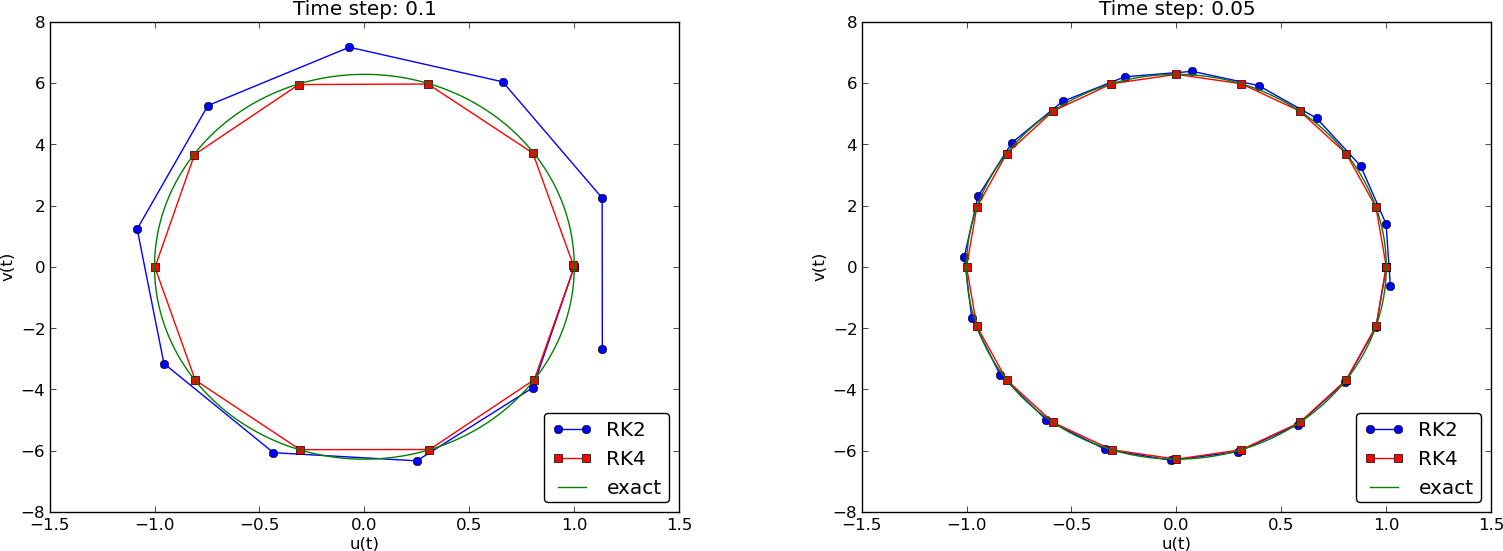Figure 11: Comparison of Runge-Kutta schemes.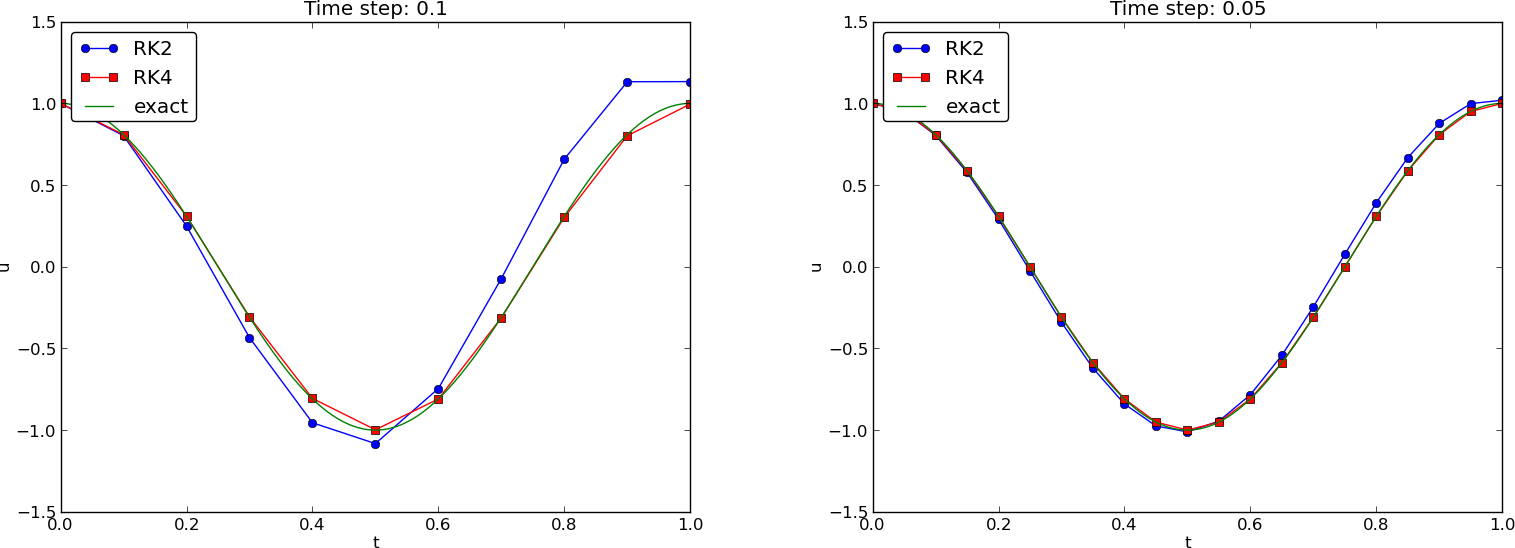The visual impression is that the 4th-order Runge-Kutta method is very accurate, under all circumstances in these tests, while the 2nd-order scheme suffers from amplitude errors unless the time step is very small.

The corresponding results for the Crank-Nicolson scheme are shown in Figure 12. It is clear that the Crank-Nicolson scheme outperforms the 2nd-order Runge-Kutta method. Both schemes have the same order of accuracy $$\Oof{\Delta t^2}$$, but their differences in the accuracy that matters in a real physical application is very clearly pronounced in this example. Exercise 1.13: Investigate the amplitude errors of many solvers invites you to investigate how the amplitude is computed by a series of famous methods for first-order ODEs.

Figure 12: Long-time behavior of the Crank-Nicolson scheme in the phase plane.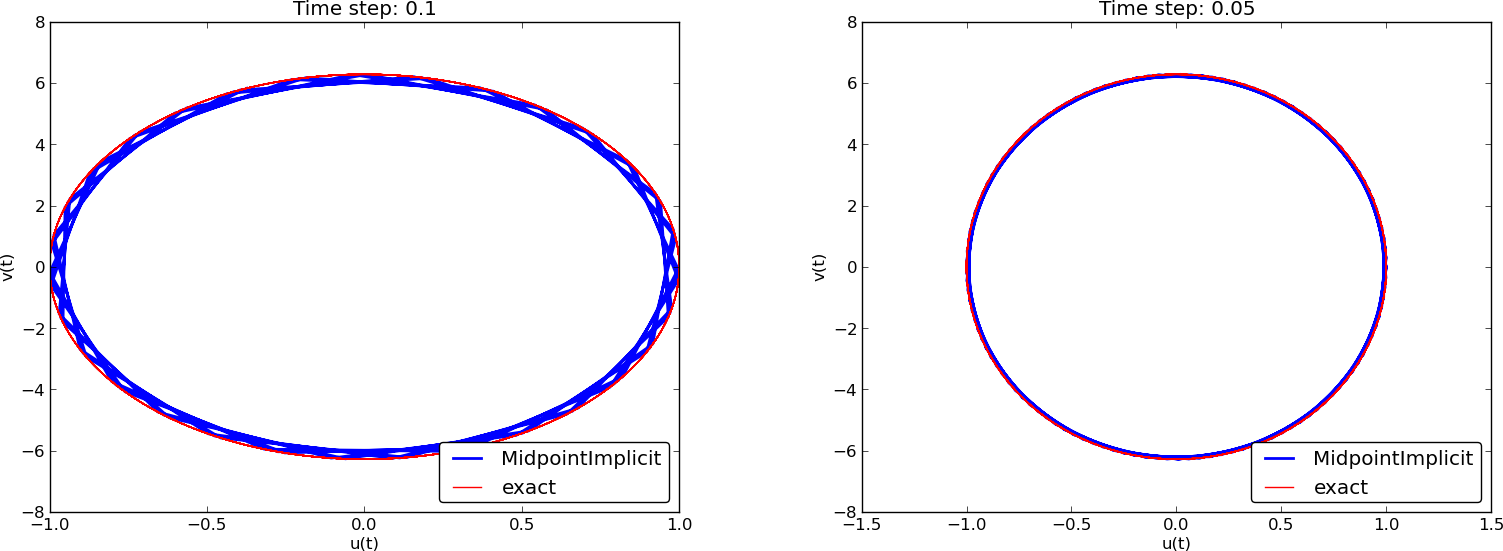## Analysis of the Forward Euler scheme

We may try to find exact solutions of the discrete equations (1.28)-(1.29) in the Forward Euler method to better understand why this otherwise useful method has so bad performance for vibration ODEs. An "ansatz" for the solution of the discrete equations is \begin{align*} u^n &= IA^n,\\ v^n &= qIA^n, \end{align*} where $$q$$ and $$A$$ are scalars to be determined. We could have used a complex exponential form $$e^{i\tilde\omega n\Delta t}$$ since we get oscillatory solutions, but the oscillations grow in the Forward Euler method, so the numerical frequency $$\tilde\omega$$ will be complex anyway (producing an exponentially growing amplitude). Therefore, it is easier to just work with potentially complex $$A$$ and $$q$$ as introduced above.

The Forward Euler scheme leads to \begin{align*} A &= 1 + \Delta t q,\\ A &= 1 - \Delta t\omega^2 q^{-1}\tp \end{align*} We can easily eliminate $$A$$, get $$q^2 + \omega^2=0$$, and solve for $$q = \pm i\omega,$$ which gives $$A = 1 \pm \Delta t i\omega\tp$$ We shall take the real part of $$A^n$$ as the solution. The two values of $$A$$ are complex conjugates, and the real part of $$A^n$$ will be the same for both roots. This is easy to realize if we rewrite the complex numbers in polar form, which is also convenient for further analysis and understanding. The polar form $$re^{i\theta}$$ of a complex number $$x+iy$$ has $$r=\sqrt{x^2+y^2}$$ and $$\theta = \tan^{-1}(y/x)$$. Hence, the polar form of the two values for $$A$$ becomes $$1 \pm \Delta t i\omega = \sqrt{1+\omega^2\Delta t^2}e^{\pm i\tan^{-1}(\omega\Delta t)}\tp$$ Now it is very easy to compute $$A^n$$: $$(1 \pm \Delta t i\omega)^n = (1+\omega^2\Delta t^2)^{n/2}e^{\pm ni\tan^{-1}(\omega\Delta t)}\tp$$ Since $$\cos (\theta n) = \cos (-\theta n)$$, the real parts of the two numbers become the same. We therefore continue with the solution that has the plus sign.

The general solution is $$u^n = CA^n$$, where $$C$$ is a constant determined from the initial condition: $$u^0=C=I$$. We have $$u^n=IA^n$$ and $$v^n=qIA^n$$. The final solutions are just the real part of the expressions in polar form: \begin{align} u^n & = I(1+\omega^2\Delta t^2)^{n/2}\cos (n\tan^{-1}(\omega\Delta t)), \tag{1.44}\\ v^n &=- \omega I(1+\omega^2\Delta t^2)^{n/2}\sin (n\tan^{-1}(\omega\Delta t))\tp \tag{1.45} \end{align} The expression $$(1+\omega^2\Delta t^2)^{n/2}$$ causes growth of the amplitude, since a number greater than one is raised to a positive exponent $$n/2$$. We can develop a series expression to better understand the formula for the amplitude. Introducing $$p=\omega\Delta t$$ as the key variable and using sympy gives

>>> from sympy import *
>>> p = symbols('p', real=True)
>>> n = symbols('n', integer=True, positive=True)
>>> amplitude = (1 + p**2)**(n/2)
>>> amplitude.series(p, 0, 4)
1 + n*p**2/2 + O(p**4)


The amplitude goes like $$1 + \half n\omega^2\Delta t^2$$, clearly growing linearly in time (with $$n$$).

We can also investigate the error in the angular frequency by a series expansion:

>>> n*atan(p).series(p, 0, 4)
n*(p - p**3/3 + O(p**4))


This means that the solution for $$u^n$$ can be written as $$u^n = (1 + \half n\omega^2\Delta t^2 + \Oof{\Delta t^4}) \cos\left(\omega t - \frac{1}{3}\omega t\Delta t^2 + \Oof{\Delta t^4}\right) \tp$$ The error in the angular frequency is of the same order as in the scheme (1.7) for the second-order ODE, but the error in the amplitude is severe.

# Energy considerations

The observations of various methods in the previous section can be better interpreted if we compute a quantity reflecting the total energy of the system. It turns out that this quantity, $$E(t) = \half(u^{\prime})^2 + \half\omega^2u^2,$$ is constant for all $$t$$. Checking that $$E(t)$$ really remains constant brings evidence that the numerical computations are sound. It turns out that $$E$$ is proportional to the mechanical energy in the system. Conservation of energy is much used to check numerical simulations, so it is well invested time to dive into this subject.

## Derivation of the energy expression

We start out with multiplying $$u^{\prime\prime} + \omega^2 u = 0,$$ by $$u^{\prime}$$ and integrating from $$0$$ to $$T$$: $$\int_0^T u^{\prime\prime}u^{\prime} dt + \int_0^T\omega^2 u u^{\prime} dt = 0\tp$$ Observing that $$u^{\prime\prime}u^{\prime} = \frac{d}{dt}\half(u^{\prime})^2,\quad uu^{\prime} = \frac{d}{dt} {\half}u^2,$$ we get $$\int_0^T (\frac{d}{dt}\half(u^{\prime})^2 + \frac{d}{dt} \half\omega^2u^2)dt = E(T) - E(0)=0,$$ where we have introduced $$\begin{equation} E(t) = \half(u^{\prime})^2 + \half\omega^2u^2\tp \tag{1.46} \end{equation}$$ The important result from this derivation is that the total energy is constant: $$E(t) = E(0)\tp$$

$$E(t)$$ is closely related to the system's energy. The quantity $$E(t)$$ derived above is physically not the mechanical energy of a vibrating mechanical system, but the energy per unit mass. To see this, we start with Newton's second law $$F=ma$$ ($$F$$ is the sum of forces, $$m$$ is the mass of the system, and $$a$$ is the acceleration). The displacement $$u$$ is related to $$a$$ through $$a=u^{\prime\prime}$$. With a spring force as the only force we have $$F=-ku$$, where $$k$$ is a spring constant measuring the stiffness of the spring. Newton's second law then implies the differential equation $$-ku = mu^{\prime\prime}\quad\Rightarrow mu^{\prime\prime} + ku = 0\tp$$ This equation of motion can be turned into an energy balance equation by finding the work done by each term during a time interval $$[0,T]$$. To this end, we multiply the equation by $$du=u^{\prime}dt$$ and integrate: $$\int_0^T muu^{\prime}dt + \int_0^T kuu^{\prime}dt = 0\tp$$ The result is $$\tilde E(t) = E_k(t) + E_p(t) = 0,$$ where $$\begin{equation} E_k(t) = \frac{1}{2}mv^2,\quad v=u^{\prime}, \tag{1.47} \end{equation}$$ is the kinetic energy of the system, and $$\begin{equation} E_p(t) = {\half}ku^2 \tag{1.48} \end{equation}$$ is the potential energy. The sum $$\tilde E(t)$$ is the total mechanical energy. The derivation demonstrates the famous energy principle that, under the right physical circumstances, any change in the kinetic energy is due to a change in potential energy and vice versa. (This principle breaks down when we introduce damping in the system, as we do in the section Generalization: damping, nonlinearities, and excitation.)

The equation $$mu^{\prime\prime}+ku=0$$ can be divided by $$m$$ and written as $$u^{\prime\prime} + \omega^2u=0$$ for $$\omega=\sqrt{k/m}$$. The energy expression $$E(t)=\half(u^{\prime})^2 + \half\omega^2u^2$$ derived earlier is then $$\tilde E(t)/m$$, i.e., mechanical energy per unit mass.

### Energy of the exact solution

Analytically, we have $$u(t)=I\cos\omega t$$, if $$u(0)=I$$ and $$u^{\prime}(0)=0$$, so we can easily check the energy evolution and confirm that $$E(t)$$ is constant: $$E(t) = {\half}I^2 (-\omega\sin\omega t)^2 + \half\omega^2 I^2 \cos^2\omega t = \half\omega^2 (\sin^2\omega t + \cos^2\omega t) = \half\omega^2 \tp$$

### Growth of energy in the Forward Euler scheme

The energy at time level $$n+1$$ in the Forward Euler scheme can easily be shown to increase: \begin{align*} E^{n+1} &= \half(v^{n+1})^2 + \half\omega^2 (u^{n+1})^2\\ &= \half(v^n - \omega^2\Delta t u^n)^2 + \half\omega^2(u^n + \Delta t v^n)^2\\ &= (1 + \Delta t^2\omega^2)E^n\tp \end{align*}

## An error measure based on energy

The constant energy is well expressed by its initial value $$E(0)$$, so that the error in mechanical energy can be computed as a mesh function by $$\begin{equation} e_E^n = \half\left(\frac{u^{n+1}-u^{n-1}}{2\Delta t}\right)^2 + \half\omega^2 (u^n)^2 - E(0), \quad n=1,\ldots,N_t-1, \tag{1.49} \end{equation}$$ where $$E(0) = {\half}V^2 + \half\omega^2I^2,$$ if $$u(0)=I$$ and $$u^{\prime}(0)=V$$. Note that we have used a centered approximation to $$u^{\prime}$$: $$u^{\prime}(t_n)\approx [D_{2t}u]^n$$.

A useful norm of the mesh function $$e_E^n$$ for the discrete mechanical energy can be the maximum absolute value of $$e_E^n$$: $$||e_E^n||_{\ell^\infty} = \max_{1\leq n < N_t} |e_E^n|\tp$$ Alternatively, we can compute other norms involving integration over all mesh points, but we are often interested in worst case deviation of the energy, and then the maximum value is of particular relevance.

A vectorized Python implementation of $$e_E^n$$ takes the form

# import numpy as np and compute u, t
dt = t-t
E = 0.5*((u[2:] - u[:-2])/(2*dt))**2 + 0.5*w**2*u[1:-1]**2
E0 = 0.5*V**2 + 0.5**w**2*I**2
e_E = E - E0
e_E_norm = np.abs(e_E).max()


The convergence rates of the quantity e_E_norm can be used for verification. The value of e_E_norm is also useful for comparing schemes through their ability to preserve energy. Below is a table demonstrating the relative error in total energy for various schemes (computed by the vib_undamped_odespy.py program). The test problem is $$u^{\prime\prime} + 4\pi^2 u =0$$ with $$u(0)=1$$ and $$u'(0)=0$$, so the period is 1 and $$E(t)\approx 4.93$$. We clearly see that the Crank-Nicolson and the Runge-Kutta schemes are superior to the Forward and Backward Euler schemes already after one period.

 Method $$T$$ $$\Delta t$$ $$\max \left\vert e_E^n\right\vert/e_E^0$$ Forward Euler $$1$$ $$0.025$$ $$1.678\cdot 10^{0}$$ Backward Euler $$1$$ $$0.025$$ $$6.235\cdot 10^{-1}$$ Crank-Nicolson $$1$$ $$0.025$$ $$1.221\cdot 10^{-2}$$ Runge-Kutta 2nd-order $$1$$ $$0.025$$ $$6.076\cdot 10^{-3}$$ Runge-Kutta 4th-order $$1$$ $$0.025$$ $$8.214\cdot 10^{-3}$$

However, after 10 periods, the picture is much more dramatic:

 Method $$T$$ $$\Delta t$$ $$\max \left\vert e_E^n\right\vert/e_E^0$$ Forward Euler $$10$$ $$0.025$$ $$1.788\cdot 10^{4}$$ Backward Euler $$10$$ $$0.025$$ $$1.000\cdot 10^{0}$$ Crank-Nicolson $$10$$ $$0.025$$ $$1.221\cdot 10^{-2}$$ Runge-Kutta 2nd-order $$10$$ $$0.025$$ $$6.250\cdot 10^{-2}$$ Runge-Kutta 4th-order $$10$$ $$0.025$$ $$8.288\cdot 10^{-3}$$

The Runge-Kutta and Crank-Nicolson methods hardly change their energy error with $$T$$, while the error in the Forward Euler method grows to huge levels and a relative error of 1 in the Backward Euler method points to $$E(t)\rightarrow 0$$ as $$t$$ grows large.

Running multiple values of $$\Delta t$$, we can get some insight into the convergence of the energy error:

 Method $$T$$ $$\Delta t$$ $$\max \left\vert e_E^n\right\vert/e_E^0$$ Forward Euler $$10$$ $$0.05$$ $$1.120\cdot 10^{8}$$ Forward Euler $$10$$ $$0.025$$ $$1.788\cdot 10^{4}$$ Forward Euler $$10$$ $$0.0125$$ $$1.374\cdot 10^{2}$$ Backward Euler $$10$$ $$0.05$$ $$1.000\cdot 10^{0}$$ Backward Euler $$10$$ $$0.025$$ $$1.000\cdot 10^{0}$$ Backward Euler $$10$$ $$0.0125$$ $$9.928\cdot 10^{-1}$$ Crank-Nicolson $$10$$ $$0.05$$ $$4.756\cdot 10^{-2}$$ Crank-Nicolson $$10$$ $$0.025$$ $$1.221\cdot 10^{-2}$$ Crank-Nicolson $$10$$ $$0.0125$$ $$3.125\cdot 10^{-3}$$ Runge-Kutta 2nd-order $$10$$ $$0.05$$ $$6.152\cdot 10^{-1}$$ Runge-Kutta 2nd-order $$10$$ $$0.025$$ $$6.250\cdot 10^{-2}$$ Runge-Kutta 2nd-order $$10$$ $$0.0125$$ $$7.631\cdot 10^{-3}$$ Runge-Kutta 4th-order $$10$$ $$0.05$$ $$3.510\cdot 10^{-2}$$ Runge-Kutta 4th-order $$10$$ $$0.025$$ $$8.288\cdot 10^{-3}$$ Runge-Kutta 4th-order $$10$$ $$0.0125$$ $$2.058\cdot 10^{-3}$$

A striking fact from this table is that the error of the Forward Euler method is reduced by the same factor as $$\Delta t$$ is reduced by, while the error in the Crank-Nicolson method has a reduction proportional to $$\Delta t^2$$ (we cannot say anything for the Backward Euler method). However, for the RK2 method, halving $$\Delta t$$ reduces the error by almost a factor of 10 (!), and for the RK4 method the reduction seems proportional to $$\Delta t^2$$ only (and the trend is confirmed by running smaller time steps, so for $$\Delta t = 3.9\cdot 10^{-4}$$ the relative error of RK2 is a factor 10 smaller than that of RK4!).

# The Euler-Cromer method

While the Runge-Kutta methods and the Crank-Nicolson scheme work well for the vibration equation modeled as a first-order ODE system, both were inferior to the straightforward centered difference scheme for the second-order equation $$u^{\prime\prime}+\omega^2u=0$$. However, there is a similarly successful scheme available for the first-order system $$u^{\prime}=v$$, $$v^{\prime}=-\omega^2u$$, to be presented below. The ideas of the scheme and their further developments have become very popular in particle and rigid body dynamics and hence widely used by physicists.

## Forward-backward discretization

The idea is to apply a Forward Euler discretization to the first equation and a Backward Euler discretization to the second. In operator notation this is stated as \begin{align} \lbrack D_t^+u &= v\rbrack^n, \tag{1.50}\\ \lbrack D_t^-v &= -\omega^2 u\rbrack^{n+1} \tp \tag{1.51} \end{align} We can write out the formulas and collect the unknowns on the left-hand side: \begin{align} u^{n+1} &= u^n + \Delta t v^n, \tag{1.52}\\ v^{n+1} &= v^n -\Delta t \omega^2u^{n+1} \tag{1.53} \tp \end{align} We realize that after $$u^{n+1}$$ has been computed from (1.52), it may be used directly in (1.53) to compute $$v^{n+1}$$.

In physics, it is more common to update the $$v$$ equation first, with a forward difference, and thereafter the $$u$$ equation, with a backward difference that applies the most recently computed $$v$$ value: \begin{align} v^{n+1} &= v^n -\Delta t \omega^2u^{n}, \tag{1.54}\\ u^{n+1} &= u^n + \Delta t v^{n+1}\tp \tag{1.55} \end{align} The advantage of ordering the ODEs as in (1.54)-(1.55) becomes evident when considering complicated models. Such models are included if we write our vibration ODE more generally as $$u^{\prime\prime} + g(u, u^{\prime}, t)=0\tp$$ We can rewrite this second-order ODE as two first-order ODEs, \begin{align*} v^{\prime} &= -g(u,v,t),\\ u^{\prime} &= v\tp \end{align*} This rewrite allows the following scheme to be used: \begin{align*} v^{n+1} &= v^n -\Delta t\, g(u^n,v^n,t),\\ u^{n+1} &= u^n + \Delta t\, v^{n+1}\tp \end{align*} We realize that the first update works well with any $$g$$ since old values $$u^n$$ and $$v^n$$ are used. Switching the equations would demand $$u^{n+1}$$ and $$v^{n+1}$$ values in $$g$$ and result in nonlinear algebraic equations to be solved at each time level.

The scheme (1.54)-(1.55) goes under several names: forward-backward scheme, semi-implicit Euler method, semi-explicit Euler, symplectic Euler, Newton-Stoermer-Verlet, and Euler-Cromer. We shall stick to the latter name. Since both time discretizations are based on first-order difference approximation, one may think that the scheme is only of first-order, but this is not true: the use of a forward and then a backward difference make errors cancel so that the overall error in the scheme is $$\Oof{\Delta t^2}$$. This is explained below.

How does the Euler-Cromer method preserve the total energy? We may run the example from the section An error measure based on energy:

 Method $$T$$ $$\Delta t$$ $$\max \left\vert e_E^n\right\vert/e_E^0$$ Euler-Cromer $$10$$ $$0.05$$ $$2.530\cdot 10^{-2}$$ Euler-Cromer $$10$$ $$0.025$$ $$6.206\cdot 10^{-3}$$ Euler-Cromer $$10$$ $$0.0125$$ $$1.544\cdot 10^{-3}$$

The relative error in the total energy decreases as $$\Delta t^2$$, and the error level is slightly lower than for the Crank-Nicolson and Runge-Kutta methods.

## Equivalence with the scheme for the second-order ODE

We shall now show that the Euler-Cromer scheme for the system of first-order equations is equivalent to the centered finite difference method for the second-order vibration ODE (!).

We may eliminate the $$v^n$$ variable from (1.52)-(1.53) or (1.54)-(1.55). The $$v^{n+1}$$ term in (1.54) can be eliminated from (1.55): $$\begin{equation} u^{n+1} = u^n + \Delta t (v^n - \omega^2\Delta t u^n)\tp \tag{1.56} \end{equation}$$ The $$v^{n}$$ quantity can be expressed by $$u^n$$ and $$u^{n-1}$$ using (1.55): $$v^{n} = \frac{u^n - u^{n-1}}{\Delta t},$$ and when this is inserted in (1.56) we get $$\begin{equation} u^{n+1} = 2u^n - u^{n-1} - \Delta t^2 \omega^2u^{n}, \tag{1.57} \end{equation}$$ which is nothing but the centered scheme (1.7)! The two seemingly different numerical methods are mathematically equivalent. Consequently, the previous analysis of (1.7) also applies to the Euler-Cromer method. In particular, the amplitude is constant, given that the stability criterion is fulfilled, but there is always an angular frequency error (1.19). Exercise 1.18: Analysis of the Euler-Cromer scheme gives guidance on how to derive the exact discrete solution of the two equations in the Euler-Cromer method.

Although the Euler-Cromer scheme and the method (1.7) are equivalent, there could be differences in the way they handle the initial conditions. Let is look into this topic. The initial condition $$u^{\prime}=0$$ means $$u^{\prime}=v=0$$. From (1.54) we get $$v^1=v^0-\Delta t\omega^2 u^0 =\Delta t\omega^2 u^0,$$ and from (1.55) it follows that $$u^1=u^0 + \Delta t v^1 = u^0 - \omega^2\Delta t^2 u^0\tp$$ When we previously used a centered approximation of $$u^{\prime}(0)=0$$ combined with the discretization (1.7) of the second-order ODE, we got a slightly different result: $$u^1=u^0 - \frac{1}{2}\omega^2\Delta t^2 u^0$$. The difference is $$\frac{1}{2}\omega^2\Delta t^2 u^0$$, which is of second order in $$\Delta t$$, seemingly consistent with the overall error in the scheme for the differential equation model.

A different view can also be taken. If we approximate $$u^{\prime}(0)=0$$ by a backward difference, $$(u^0-u^{-1})/\Delta t =0$$, we get $$u^{-1}=u^0$$, and when combined with (1.7), it results in $$u^1=u^0 - \omega^2\Delta t^2 u^0$$. This means that the Euler-Cromer method based on (1.55)-(1.54) corresponds to using only a first-order approximation to the initial condition in the method from the section A centered finite difference scheme.

Correspondingly, using the formulation (1.52)-(1.53) with $$v^n=0$$ leads to $$u^1=u^0$$, which can be interpreted as using a forward difference approximation for the initial condition $$u^{\prime}(0)=0$$. Both Euler-Cromer formulations lead to slightly different values for $$u^1$$ compared to the method in the section A centered finite difference scheme. The error is $$\frac{1}{2}\omega^2\Delta t^2 u^0$$.

## Implementation

### Solver function

The function below, found in vib_undamped_EulerCromer.py, implements the Euler-Cromer scheme (1.54)-(1.55):

import numpy as np

def solver(I, w, dt, T):
"""
Solve v' = - w**2*u, u'=v for t in (0,T], u(0)=I and v(0)=0,
by an Euler-Cromer method.
"""
dt = float(dt)
Nt = int(round(T/dt))
u = np.zeros(Nt+1)
v = np.zeros(Nt+1)
t = np.linspace(0, Nt*dt, Nt+1)

v = 0
u = I
for n in range(0, Nt):
v[n+1] = v[n] - dt*w**2*u[n]
u[n+1] = u[n] + dt*v[n+1]
return u, v, t


### Verification

Since the Euler-Cromer scheme is equivalent to the finite difference method for the second-order ODE $$u^{\prime\prime}+\omega^2u=0$$ (see the section Equivalence with the scheme for the second-order ODE), the performance of the above solver function is the same as for the solver function in the section Implementation. The only difference is the formula for the first time step, as discussed above. This deviation in the Euler-Cromer scheme means that the discrete solution listed in the section Exact discrete solution is not a solution of the Euler-Cromer scheme!

To verify the implementation of the Euler-Cromer method we can adjust v so that the computer-generated values can be compared with the formula (1.20) from in the section Exact discrete solution. This adjustment is done in an alternative solver function, solver_ic_fix in vib_EulerCromer.py. Since we now have an exact solution of the discrete equations available, we can write a test function test_solver for checking the equality of computed values with the formula (1.20):

def test_solver():
"""
Test solver with fixed initial condition against
equivalent scheme for the 2nd-order ODE u'' + u = 0.
"""
I = 1.2; w = 2.0; T = 5
dt = 2/w  # longest possible time step
u, v, t = solver_ic_fix(I, w, dt, T)
from vib_undamped import solver as solver2  # 2nd-order ODE
u2, t2 = solver2(I, w, dt, T)
error = np.abs(u - u2).max()
tol = 1E-14
assert error < tol


Another function, demo, visualizes the difference between the Euler-Cromer scheme and the scheme (1.7) for the second-oder ODE, arising from the mismatch in the first time level.

### Using Odespy

The Euler-Cromer method is also available in the Odespy package. The important thing to remember, when using this implementation, is that we must order the unknowns as $$v$$ and $$u$$, so the u vector at each time level consists of the velocity $$v$$ as first component and the displacement $$u$$ as second component:

# Define ODE
def f(u, t, w=1):
v, u = u
return [-w**2*u, v]

# Initialize solver
I = 1
w = 2*np.pi
import odespy
solver = odespy.EulerCromer(f, f_kwargs={'w': w})
solver.set_initial_condition([0, I])

# Compute time mesh
P = 2*np.pi/w  # duration of one period
dt = P/timesteps_per_period
Nt = num_periods*timesteps_per_period
T = Nt*dt
import numpy as np
t_mesh = np.linspace(0, T, Nt+1)

# Solve ODE
u, t = solver.solve(t_mesh)
u = u[:,1]  # Extract displacement


### Convergence rates

We may use the convergence_rates function in the file vib_undamped.py to investigate the convergence rate of the Euler-Cromer method, see the convergence_rate function in the file vib_undamped_EulerCromer.py. Since we could eliminate $$v$$ to get a scheme for $$u$$ that is equivalent to the finite difference method for the second-order equation in $$u$$, we would expect the convergence rates to be the same, i.e., $$\mathcal{O}(\Delta t^2)$$. However, measuring the convergence rate of $$u$$ in the Euler-Cromer scheme shows that it is $$\mathcal{O}(\Delta t)$$! Adjusting the initial condition does not change the rate. Adjusting $$\omega$$, as outlined in the section The error in the numerical frequency, gives a 4th-order method there, while there is no increase in the measured rate in the Euler-Cromer scheme. It is obvious that the Euler-Cromer scheme is dramatically much better than the two other first-order methods, Forward Euler and Backward Euler, but this is not reflected in the convergence rate of $$u$$.

## The Stoermer-Verlet algorithm

Another very popular algorithm for vibration problems, especially for long time simulations, is the Stoermer-Verlet algorithm. It has become the method among physicists for molecular simulations as well as particle and rigid body dynamics.

The method can be derived by applying the Euler-Cromer idea twice, in a symmetric fashion, during the interval $$[t_n,t_{n+1}]$$:

1. solve $$v^{\prime}=-\omega u$$ by a Forward Euler step in $$[t_n,t_{n+\half}]$$
2. solve $$u^{\prime}=v$$ by a Backward Euler step in $$[t_n,t_{n+\half}]$$
3. solve $$u^{\prime}=v$$ by a Forward Euler step in $$[t_{n+\half}, t_{n+1}]$$
4. solve $$v^{\prime}=-\omega u$$ by a Backward Euler step in $$[t_{n+\half}, t_{n+1}]$$
With mathematics, \begin{align*} \frac{v^{n+\half}-v^n}{\half\Delta t} &= -\omega^2 u^n,\\ \frac{u^{n+\half}-u^n}{\half\Delta t} &= v^{n+\half},\\ \frac{u^{n+1}-u^{n-\half}}{\half\Delta t} &= v^{n+\half},\\ \frac{v^{n+1}-v^{n+\half}}{\half\Delta t} &= -\omega^2 u^{n+1}\tp \end{align*} The two steps in the middle can be combined to $$\frac{u^{n+1}-u^{n-1}}{\Delta t} = v^{n+\half},$$ and consequently \begin{align} v^{n+\half} &= v^n - \half\Delta t \omega^2 u^n, \tag{1.58}\\ u^{n+1} &= u^{n} + \Delta t v^{n+\half}, \tag{1.59}\\ v^{n+1} &= v^{n+\half} - \half\Delta t \omega^2 u^{n+1}\tp \tag{1.60} \end{align} Writing the last equation as $$v^n = v^{n-\half} - \half\Delta t\omega^2 u^n$$ and using this $$v^n$$ in the first equation gives $$v^{n+\half} = v^{n-\half} - \Delta t\omega^2 u^n$$, and the scheme can be written as two steps: \begin{align} v^{n+\half} &= v^{n-\half} - \Delta t \omega^2 u^n, \tag{1.61}\\ u^{n+1} &= u^{n} + \Delta t v^{n+\half}, \tag{1.62} \end{align} which is nothing but straightforward centered differences for the $$2\times 2$$ ODE system on a staggered mesh, see the section The Euler-Cromer scheme on a staggered mesh. We have thus seen that four different reasonings (discretizing $$u^{\prime\prime}+\omega^2 u$$ directly, using Euler-Cromer, using Stoermer-Verlet, and using centered differences for the $$2times 2$$ system on a staggered mesh) all end up with the same equations! The main difference is that the traditional Euler-Cromer displays first-order convergence in $$\Delta t$$ (due to less symmetry in the way $$u$$ and $$v$$ are treated) while the others are $$\Oof{\Delta t^2}$$ schemes.

The most numerical stable scheme, with respect to accumulation of rounding errors, is (1.61)-(1.62). It has, according to , better properties in this regard than the direct scheme for the second-order ODE.

# Staggered mesh

A more intuitive discretization than the Euler-Cromer method, yet equivalent, employs solely centered differences in a natural way for the $$2\times 2$$ first-order ODE system. The scheme is in fact fully equivalent to the second-order scheme for $$u''+\omega u=0$$, also for the first time step. Such a scheme needs to operate on a staggered mesh in time. Staggered meshes are very popular in many physical application, maybe foremost fluid dynamics and electromagnetics, so the topic is important to learn.

## The Euler-Cromer scheme on a staggered mesh

In a staggered mesh, the unknowns are sought at different points in the mesh. Specifically, $$u$$ is sought at integer time points $$t_n$$ and $$v$$ is sought at $$t_{n+1/2}$$ between two $$u$$ points. The unknowns are then $$u^1, v^{3/2}, u^2, v^{5/2}$$, and so on. We typically use the notation $$u^n$$ and $$v^{n+\half}$$ for the two unknown mesh functions. Figure 13 presents a graphical sketch of two mesh functions $$u$$ and $$v$$ on a staggered mesh.

Figure 13: Examples on mesh functions on a staggered mesh in time.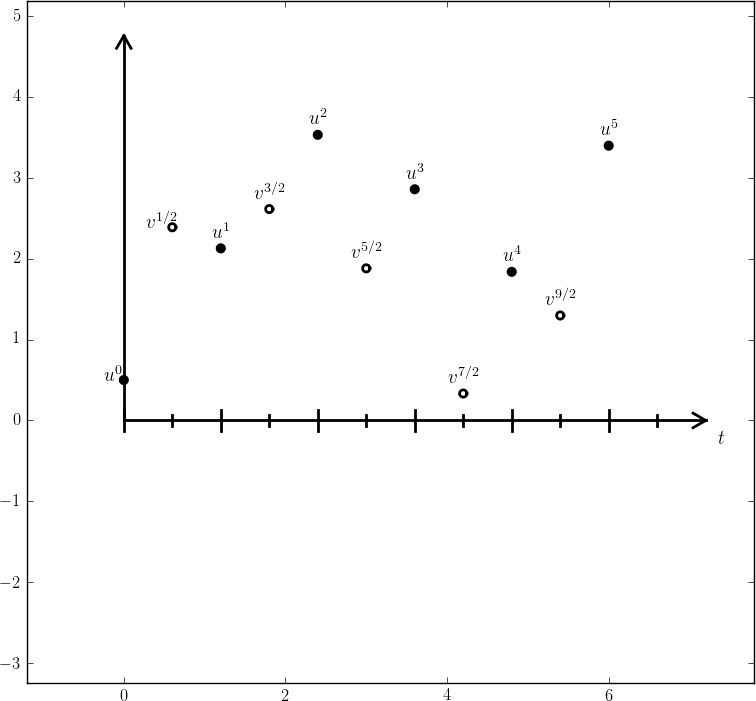On a staggered mesh it is natural to use centered difference approximations, expressed in operator notation as \begin{align} \lbrack D_t u &= v\rbrack^{n+\half}, \tag{1.63}\\ \lbrack D_t v &= -\omega u\rbrack^{n+1} \tp \tag{1.64} \end{align} or if we switch the sequence of the equations: \begin{align} \lbrack D_t v &= -\omega u\rbrack^{n}, \tag{1.65}\\ \lbrack D_t u &= v\rbrack^{n+\half} \tp \tag{1.66} \end{align} Writing out the formulas gives \begin{align} v^{n+\frac{1}{2}} &= v^{n-\half} -\Delta t \omega^2u^{n} \tag{1.67},\\ u^{n+1} &= u^{n} + \Delta t v^{n+\half} \tag{1.68} \tp \end{align}

We can eliminate the $$v$$ values and get back the centered scheme based on the second-order differential equation $$u^{\prime\prime} +\omega^2 u = 0$$, so all these three schemes are equivalent. However, they differ somewhat in the treatment of the initial conditions.

Suppose we have $$u(0)=I$$ and $$u'(0)=v(0)=0$$ as mathematical initial conditions. This means $$u^0=I$$ and $$v(0)\approx \half(v^{-\half} + v^{\half}) = 0, \quad\Rightarrow\quad v^{-\half} =- v^\half\tp$$ Using the discretized equation (1.67) for $$n=0$$ yields $$v^\half = v^{-\half} -\Delta t\omega^2 I,$$ and eliminating $$v^{-\half} =- v^{\half}$$ results in $$v^\half = -\half\Delta t\omega^2I,$$ and $$u^1 = u^0 - \half\Delta t^2\omega^2 I,$$ which is exactly the same equation for $$u^1$$ as we had in the centered scheme based on the second-order differential equation (and hence corresponds to a centered difference approximation of the initial condition for $$u'(0)$$). The conclusion is that a staggered mesh is fully equivalent with that scheme, while the forward-backward version gives a slight deviation in the computation of $$u^1$$.

We can redo the derivation of the initial conditions when $$u'(0)=V$$: $$v(0)\approx \half(v^{-\half} + v^{\half}) = V, \quad\Rightarrow\quad v^{-\half} = 2V - v^\half\tp$$ Using this $$v^{-\half}$$ in $$v^\half = v^{-\half} -\Delta t\omega^2 I,$$ then gives $$v^\half = V - \half\Delta t\omega^2 I$$. The general initial conditions are therefore \begin{align} u^0 &= I, \tag{1.69}\\ v^\half &= V - \half\Delta t\omega^2I \tag{1.70}\tp \end{align}

## Implementation of the scheme on a staggered mesh

The algorithm goes like this:

1. Set the initial values (1.69) and (1.70).
2. For $$n=1,2,\ldots$$:
1. Compute $$u^{n}$$ from (1.68).
2. Compute $$v^{n+\half}$$ from (1.67).

### Implementation with integer indices

Translating the schemes (1.68) and (1.67) to computer code faces the problem of how to store and access $$v^{n+\half}$$, since arrays only allow integer indices with base 0. We must then introduce a convention: $$v^{1+\half}$$ is stored in v[n] while $$v^{1-\half}$$ is stored in v[n-1]. We can then write the algorithm in Python as

def solver(I, w, dt, T):
dt = float(dt)
Nt = int(round(T/dt))
u = zeros(Nt+1)
v = zeros(Nt+1)
t = linspace(0, Nt*dt, Nt+1)  # mesh for u
t_v = t + dt/2                # mesh for v

u = I
v = 0 - 0.5*dt*w**2*u
for n in range(1, Nt+1):
u[n] = u[n-1] + dt*v[n-1]
v[n] = v[n-1] - dt*w**2*u[n]
return u, t, v, t_v


Note that $$u$$ and $$v$$ are returned together with the mesh points such that the complete mesh function for $$u$$ is described by u and t, while v and t_v represent the mesh function for $$v$$.

### Implementation with half-integer indices

Some prefer to see a closer relationship between the code and the mathematics for the quantities with half-integer indices. For example, we would like to replace the updating equation for v[n] by

v[n+half] = v[n-half] - dt*w**2*u[n]


This is easy to do if we could be sure that n+half means n and n-half means n-1. A possible solution is to define half as a special object such that an integer plus half results in the integer, while an integer minus half equals the integer minus 1. A simple Python class may realize the half object:

class HalfInt:
return other

def __rsub__(self, other):
return other - 1

half = HalfInt()


The __radd__ function is invoked for all expressions n+half ("right add" with self as half and other as n). Similarly, the __rsub__ function is invoked for n-half and results in n-1.

Using the half object, we can implement the algorithms in an even more readable way:

def solver(I, w, dt, T):
"""
Solve u'=v, v' = - w**2*u for t in (0,T], u(0)=I and v(0)=0,
by a central finite difference method with time step dt on
a staggered mesh with v as unknown at (i+1/2)*dt time points.
"""
dt = float(dt)
Nt = int(round(T/dt))
u = zeros(Nt+1)
v = zeros(Nt+1)
t = linspace(0, Nt*dt, Nt+1)  # mesh for u
t_v = t + dt/2                # mesh for v

u = I
v[0+half] = 0 - 0.5*dt*w**2*u
for n in range(1, Nt+1):
u[n] = u[n-1] + dt*v[n-half]
v[n+half] = v[n-half] - dt*w**2*u[n]
return u, t, v[:-1], t_v[:-1]


Verification of this code is easy as we can just compare the computed u with the u produced by the solver function in vib_undamped.py (which solves $$u''+\omega^2u=0$$ directly). The values should coincide to machine precision since the two numerical methods are mathematically equivalent. We refer to the file vib_undamped_staggered.py for the details of a unit test (test_staggered) that checks this property.

# Exercises and Problems

## Problem 1.1: Use linear/quadratic functions for verification

Consider the ODE problem $$u^{\prime\prime} + \omega^2u=f(t), \quad u(0)=I,\ u^{\prime}(0)=V,\ t\in(0,T]\tp$$

a) Discretize this equation according to $$[D_tD_t u + \omega^2 u = f]^n$$ and derive the equation for the first time step ($$u^1$$).

b) For verification purposes, we use the method of manufactured solutions (MMS) with the choice of $$\uex(t)= ct+d$$. Find restrictions on $$c$$ and $$d$$ from the initial conditions. Compute the corresponding source term $$f$$. Show that $$[D_tD_t t]^n=0$$ and use the fact that the $$D_tD_t$$ operator is linear, $$[D_tD_t (ct+d)]^n = c[D_tD_t t]^n + [D_tD_t d]^n = 0$$, to show that $$\uex$$ is also a perfect solution of the discrete equations.

c) Use sympy to do the symbolic calculations above. Here is a sketch of the program vib_undamped_verify_mms.py:

import sympy as sym
V, t, I, w, dt = sym.symbols('V t I w dt')  # global symbols
f = None  # global variable for the source term in the ODE

def ode_source_term(u):
"""Return the terms in the ODE that the source term
must balance, here u'' + w**2*u.
u is symbolic Python function of t."""
return sym.diff(u(t), t, t) + w**2*u(t)

def residual_discrete_eq(u):
"""Return the residual of the discrete eq. with u inserted."""
R = ...
return sym.simplify(R)

def residual_discrete_eq_step1(u):
"""Return the residual of the discrete eq. at the first
step with u inserted."""
R = ...
return sym.simplify(R)

def DtDt(u, dt):
"""Return 2nd-order finite difference for u_tt.
u is a symbolic Python function of t.
"""
return ...

def main(u):
"""
Given some chosen solution u (as a function of t, implemented
as a Python function), use the method of manufactured solutions
to compute the source term f, and check if u also solves
the discrete equations.
"""
print '=== Testing exact solution: %s ===' % u
print "Initial conditions u(0)=%s, u'(0)=%s:" % \
(u(t).subs(t, 0), sym.diff(u(t), t).subs(t, 0))

# Method of manufactured solution requires fitting f
global f  # source term in the ODE
f = sym.simplify(ode_lhs(u))

# Residual in discrete equations (should be 0)
print 'residual step1:', residual_discrete_eq_step1(u)
print 'residual:', residual_discrete_eq(u)

def linear():
main(lambda t: V*t + I)

if __name__ == '__main__':
linear()


Fill in the various functions such that the calls in the main function works.

d) The purpose now is to choose a quadratic function $$\uex = bt^2 + ct + d$$ as exact solution. Extend the sympy code above with a function quadratic for fitting f and checking if the discrete equations are fulfilled. (The function is very similar to linear.)

e) Will a polynomial of degree three fulfill the discrete equations?

f) Implement a solver function for computing the numerical solution of this problem.

g) Write a test function for checking that the quadratic solution is computed correctly (to machine precision, but the round-off errors accumulate and increase with $$T$$) by the solver function.

Filename: vib_undamped_verify_mms.

## Exercise 1.2: Show linear growth of the phase with time

Consider an exact solution $$I\cos (\omega t)$$ and an approximation $$I\cos(\tilde\omega t)$$. Define the phase error as the time lag between the peak $$I$$ in the exact solution and the corresponding peak in the approximation after $$m$$ periods of oscillations. Show that this phase error is linear in $$m$$.

Filename: vib_phase_error_growth.

## Exercise 1.3: Improve the accuracy by adjusting the frequency

According to (1.19), the numerical frequency deviates from the exact frequency by a (dominating) amount $$\omega^3\Delta t^2/24 >0$$. Replace the w parameter in the algorithm in the solver function in vib_undamped.py by w*(1 - (1./24)*w**2*dt**2 and test how this adjustment in the numerical algorithm improves the accuracy (use $$\Delta t =0.1$$ and simulate for 80 periods, with and without adjustment of $$\omega$$).

Filename: vib_adjust_w.

## Exercise 1.4: See if adaptive methods improve the phase error

Adaptive methods for solving ODEs aim at adjusting $$\Delta t$$ such that the error is within a user-prescribed tolerance. Implement the equation $$u^{\prime\prime}+u=0$$ in the Odespy software. Use the example on adaptive schemes in . Run the scheme with a very low tolerance (say $$10^{-14}$$) and for a long time, check the number of time points in the solver's mesh (len(solver.t_all)), and compare the phase error with that produced by the simple finite difference method from the section A centered finite difference scheme with the same number of (equally spaced) mesh points. The question is whether it pays off to use an adaptive solver or if equally many points with a simple method gives about the same accuracy.

Filename: vib_undamped_adaptive.

## Exercise 1.5: Use a Taylor polynomial to compute $$u^1$$

As an alternative to computing $$u^1$$ by (1.8), one can use a Taylor polynomial with three terms: $$u(t_1) \approx u(0) + u^{\prime}(0)\Delta t + {\half}u^{\prime\prime}(0)\Delta t^2$$ With $$u^{\prime\prime}=-\omega^2 u$$ and $$u^{\prime}(0)=0$$, show that this method also leads to (1.8). Generalize the condition on $$u^{\prime}(0)$$ to be $$u^{\prime}(0)=V$$ and compute $$u^1$$ in this case with both methods.

Filename: vib_first_step.

## Problem 1.6: Derive and investigate the velocity Verlet method

The velocity Verlet method for $$u^{\prime\prime} + \omega^2u=0$$ is based on the following ideas:

1. step $$u$$ forward from $$t_n$$ to $$t_{n+1}$$ using a three-term Taylor series,
2. replace $$u^{\prime\prime}$$ by $$-\omega^2u$$
3. discretize $$v^{\prime}=-\omega^2u$$ by a Crank-Nicolson method.
Derive the scheme, implement it, and determine empirically the convergence rate.

## Problem 1.7: Find the minimal resolution of an oscillatory function

Sketch the function on a given mesh which has the highest possible frequency. That is, this oscillatory "cos-like" function has its maxima and minima at every two grid points. Find an expression for the frequency of this function, and use the result to find the largest relevant value of $$\omega\Delta t$$ when $$\omega$$ is the frequency of an oscillating function and $$\Delta t$$ is the mesh spacing.

Filename: vib_largest_wdt.

## Exercise 1.8: Visualize the accuracy of finite differences for a cosine function

We introduce the error fraction $$E = \frac{[D_tD_t u]^n}{u^{\prime\prime}(t_n)}$$ to measure the error in the finite difference approximation $$D_tD_tu$$ to $$u^{\prime\prime}$$. Compute $$E$$ for the specific choice of a cosine/sine function of the form $$u=\exp{(i\omega t)}$$ and show that $$E = \left(\frac{2}{\omega\Delta t}\right)^2 \sin^2(\frac{\omega\Delta t}{2}) \tp$$ Plot $$E$$ as a function of $$p=\omega\Delta t$$. The relevant values of $$p$$ are $$[0,\pi]$$ (see Problem 1.7: Find the minimal resolution of an oscillatory function for why $$p>\pi$$ does not make sense). The deviation of the curve from unity visualizes the error in the approximation. Also expand $$E$$ as a Taylor polynomial in $$p$$ up to fourth degree (use, e.g., sympy).

Filename: vib_plot_fd_exp_error.

## Exercise 1.9: Verify convergence rates of the error in energy

We consider the ODE problem $$u^{\prime\prime} + \omega^2u=0$$, $$u(0)=I$$, $$u^{\prime}(0)=V$$, for $$t\in (0,T]$$. The total energy of the solution $$E(t)=\half(u^{\prime})^2 + \half\omega^2 u^2$$ should stay constant. The error in energy can be computed as explained in the section Energy considerations.

Make a test function in a separate file, where code from vib_undamped.py is imported, but the convergence_rates and test_convergence_rates functions are copied and modified to also incorporate computations of the error in energy and the convergence rate of this error. The expected rate is 2, just as for the solution itself.

Filename: test_error_conv.

## Exercise 1.10: Use linear/quadratic functions for verification

This exercise is a generalization of Problem 1.1: Use linear/quadratic functions for verification to the extended model problem (1.71) where the damping term is either linear or quadratic. Solve the various subproblems and see how the results and problem settings change with the generalized ODE in case of linear or quadratic damping. By modifying the code from Problem 1.1: Use linear/quadratic functions for verification, sympy will do most of the work required to analyze the generalized problem.

Filename: vib_verify_mms.

## Exercise 1.11: Use an exact discrete solution for verification

Write a test function in a separate file that employs the exact discrete solution (1.20) to verify the implementation of the solver function in the file vib_undamped.py.

Filename: test_vib_undamped_exact_discrete_sol.

## Exercise 1.12: Use analytical solution for convergence rate tests

The purpose of this exercise is to perform convergence tests of the problem (1.71) when $$s(u)=cu$$, $$F(t)=A\sin\phi t$$ and there is no damping. Find the complete analytical solution to the problem in this case (most textbooks on mechanics or ordinary differential equations list the various elements you need to write down the exact solution, or you can use symbolic tools like sympy or wolframalpha.com). Modify the convergence_rate function from the vib_undamped.py program to perform experiments with the extended model. Verify that the error is of order $$\Delta t^2$$.

Filename: vib_conv_rate.

## Exercise 1.13: Investigate the amplitude errors of many solvers

Use the program vib_undamped_odespy.py from the section Comparison of schemes (utilize the function amplitudes) to investigate how well famous methods for 1st-order ODEs can preserve the amplitude of $$u$$ in undamped oscillations. Test, for example, the 3rd- and 4th-order Runge-Kutta methods (RK3, RK4), the Crank-Nicolson method (CrankNicolson), the 2nd- and 3rd-order Adams-Bashforth methods (AdamsBashforth2, AdamsBashforth3), and a 2nd-order Backwards scheme (Backward2Step). The relevant governing equations are listed in the beginning of the section Alternative schemes based on 1st-order equations.

Running the code, we get the plots seen in Figure 14, 15, and 16. They show that RK4 is superior to the others, but that also CrankNicolson performs well. In fact, with RK4 the amplitude changes by less than $$0.1$$ per cent over the interval.

Figure 14: The amplitude as it changes over 100 periods for RK3 and RK4.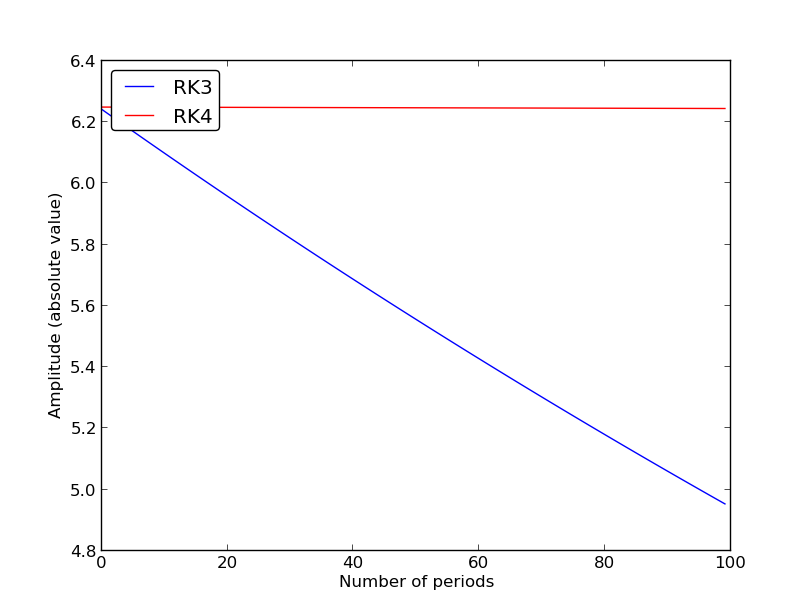Figure 15: The amplitude as it changes over 100 periods for Crank-Nicolson and Backward 2 step.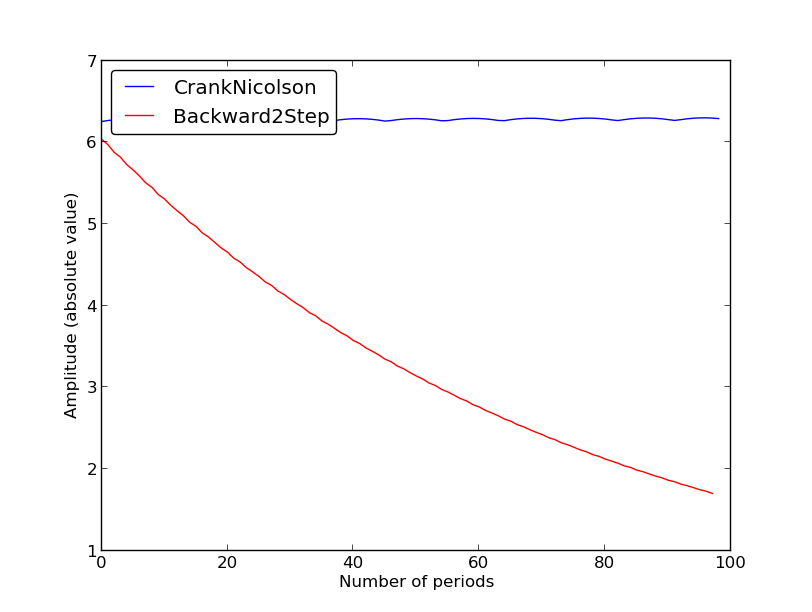Figure 16: The amplitude as it changes over 100 periods for Adams-Bashforth 2 and 3.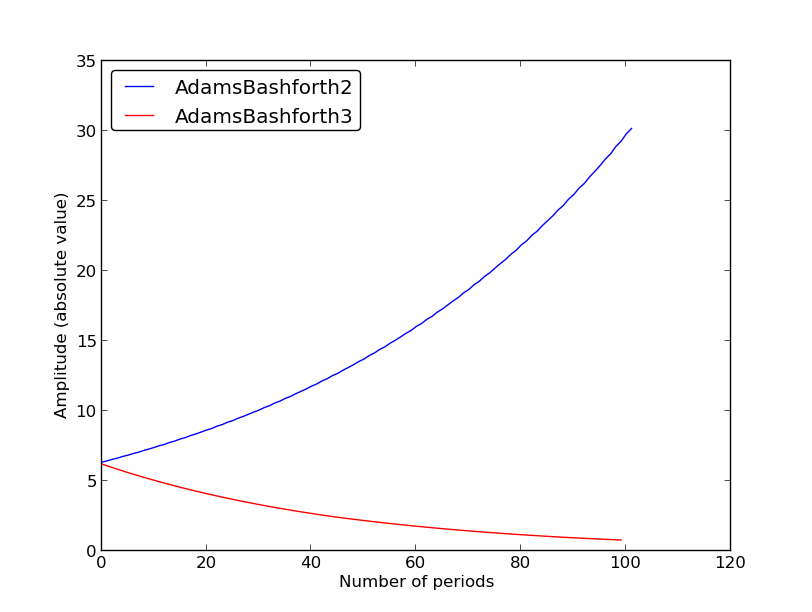Filename: vib_amplitude_errors.

## Problem 1.14: Minimize memory usage of a simple vibration solver

We consider the model problem $$u''+\omega^2 u = 0$$, $$u(0)=I$$, $$u'(0)=V$$, solved by a second-order finite difference scheme. A standard implementation typically employs an array u for storing all the $$u^n$$ values. However, at some time level n+1 where we want to compute u[n+1], all we need of previous u values are from level n and n-1. We can therefore avoid storing the entire array u, and instead work with u[n+1], u[n], and u[n-1], named as u, u_n, u_nmp1, for instance. Another possible naming convention is u, u_n, u_n[-1]. Store the solution in a file for later visualization. Make a test function that verifies the implementation by comparing with the another code for the same problem.

Filename: vib_memsave0.

## Problem 1.15: Minimize memory usage of a general vibration solver

The program vib.py stores the complete solution $$u^0,u^1,\ldots,u^{N_t}$$ in memory, which is convenient for later plotting. Make a memory minimizing version of this program where only the last three $$u^{n+1}$$, $$u^n$$, and $$u^{n-1}$$ values are stored in memory under the names u, u_n, and u_nm1 (this is the naming convention used in this book). Write each computed $$(t_{n+1}, u^{n+1})$$ pair to file. Visualize the data in the file (a cool solution is to read one line at a time and plot the $$u$$ value using the line-by-line plotter in the visualize_front_ascii function - this technique makes it trivial to visualize very long time simulations).

Filename: vib_memsave.

## Exercise 1.16: Implement the Euler-Cromer scheme for the generalized model

We consider the generalized model problem $$mu'' + f(u') + s(u) = F(t),\quad u(0)=I,\ u'(0)=V\tp$$

a) Implement the Euler-Cromer method from the section The Euler-Cromer scheme for the generalized model.

b) We expect the Euler-Cromer method to have first-order convergence rate. Make a unit test based on this expectation.

c) Consider a system with $$m=4$$, $$f(v)=b|v|v$$, $$b=0.2$$, $$s=2u$$, $$F=0$$. Compute the solution using the centered difference scheme from the section A centered scheme for linear damping and the Euler-Cromer scheme for the longest possible time step $$\Delta t$$. We can use the result from the case without damping, i.e., the largest $$\Delta t= 2/\omega$$, $$\omega\approx \sqrt{0.5}$$ in this case, but since $$b$$ will modify the frequency, we take the longest possible time step as a safety factor 0.9 times $$2/\omega$$. Refine $$\Delta t$$ three times by a factor of two and compare the two curves.

Filename: vib_EulerCromer.

## Problem 1.17: Interpret $$[D_tD_t u]^n$$ as a forward-backward difference

Show that the difference $$[D_t D_tu]^n$$ is equal to $$[D_t^+D_t^-u]^n$$ and $$D_t^-D_t^+u]^n$$. That is, instead of applying a centered difference twice one can alternatively apply a mixture of forward and backward differences.

Filename: vib_DtDt_fw_bw.

## Exercise 1.18: Analysis of the Euler-Cromer scheme

The Euler-Cromer scheme for the model problem $$u^{\prime\prime} + \omega^2 u =0$$, $$u(0)=I$$, $$u^{\prime}(0)=0$$, is given in (1.55)-(1.54). Find the exact discrete solutions of this scheme and show that the solution for $$u^n$$ coincides with that found in the section Analysis of the numerical scheme.

Hint.

Use an "ansatz" $$u^n=I\exp{(i\tilde\omega\Delta t\,n)}$$ and $$v^n=qu^n$$, where $$\tilde\omega$$ and $$q$$ are unknown parameters. The following formula is handy: $$\e^{i\tilde\omega\Delta t} + e^{i\tilde\omega(-\Delta t)} - 2 = 2\left(\cosh(i\tilde\omega\Delta t) -1 \right) =-4\sin^2(\frac{\tilde\omega\Delta t}{2})\tp$$